Скачать презентацию Chapter 14 Waiting Lines and Queuing Theory Models

ab229c8964bb05754ed82077542cd4f3.ppt

• Количество слайдов: 74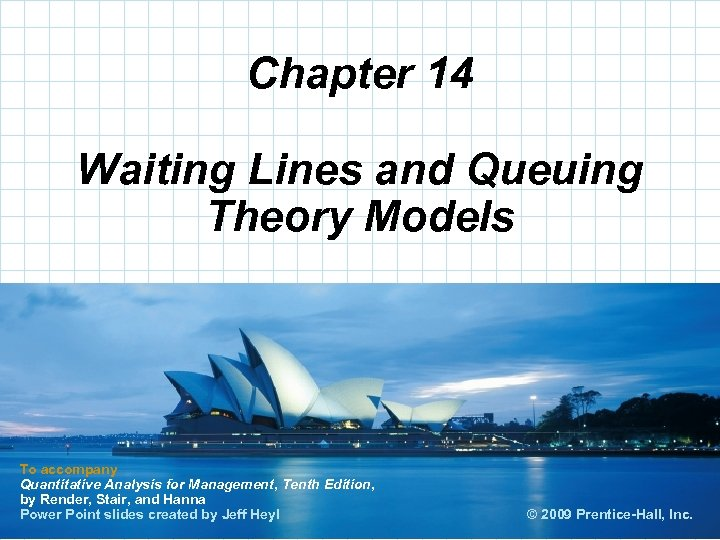Chapter 14 Waiting Lines and Queuing Theory Models To accompany Quantitative Analysis for Management, Tenth Edition, by Render, Stair, and Hanna Power Point slides created by Jeff Heyl © 2008 Prentice-Hall, Inc. © 2009 Prentice-Hall, Inc.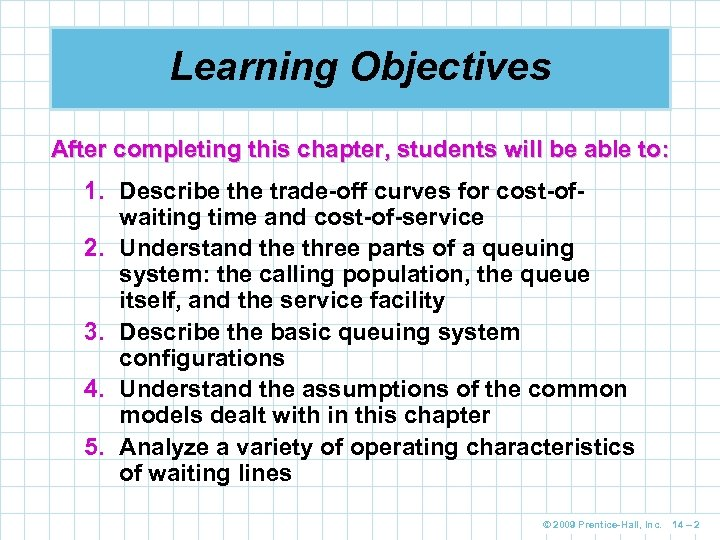Learning Objectives After completing this chapter, students will be able to: 1. Describe the trade-off curves for cost-ofwaiting time and cost-of-service 2. Understand the three parts of a queuing system: the calling population, the queue itself, and the service facility 3. Describe the basic queuing system configurations 4. Understand the assumptions of the common models dealt with in this chapter 5. Analyze a variety of operating characteristics of waiting lines © 2009 Prentice-Hall, Inc. 14 – 2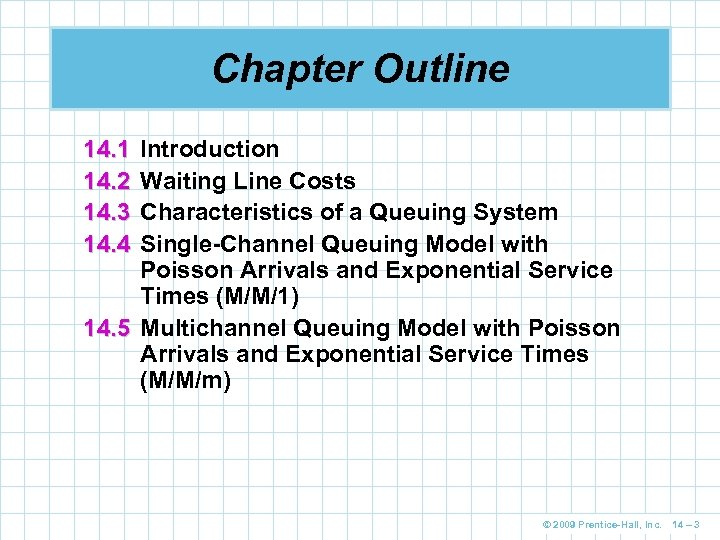Chapter Outline 14. 1 14. 2 14. 3 14. 4 Introduction Waiting Line Costs Characteristics of a Queuing System Single-Channel Queuing Model with Poisson Arrivals and Exponential Service Times (M/M/1) 14. 5 Multichannel Queuing Model with Poisson Arrivals and Exponential Service Times (M/M/m) © 2009 Prentice-Hall, Inc. 14 – 3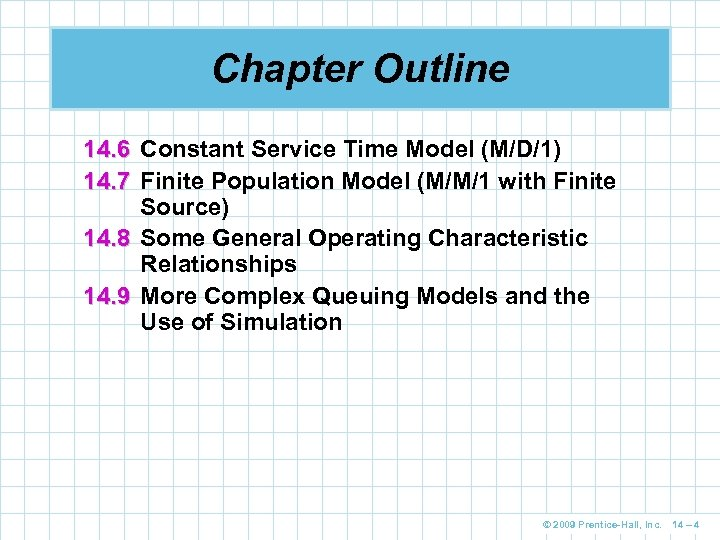Chapter Outline 14. 6 Constant Service Time Model (M/D/1) 14. 7 Finite Population Model (M/M/1 with Finite Source) 14. 8 Some General Operating Characteristic Relationships 14. 9 More Complex Queuing Models and the Use of Simulation © 2009 Prentice-Hall, Inc. 14 – 4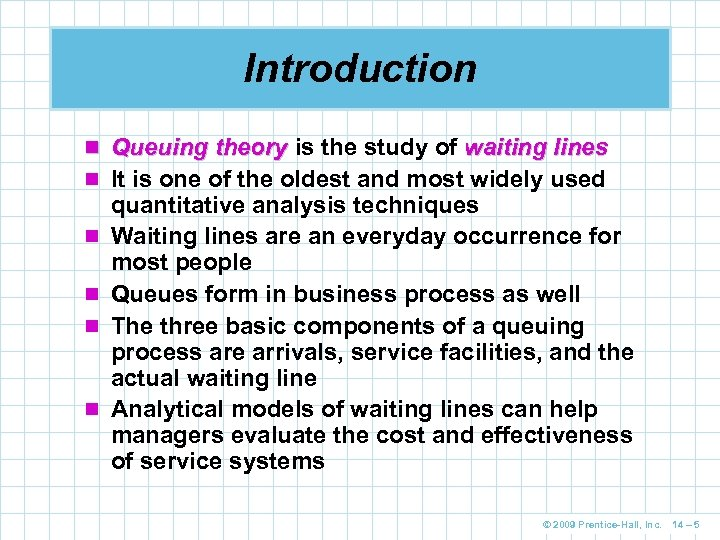Introduction n Queuing theory is the study of waiting lines n It is one of the oldest and most widely used n n quantitative analysis techniques Waiting lines are an everyday occurrence for most people Queues form in business process as well The three basic components of a queuing process are arrivals, service facilities, and the actual waiting line Analytical models of waiting lines can help managers evaluate the cost and effectiveness of service systems © 2009 Prentice-Hall, Inc. 14 – 5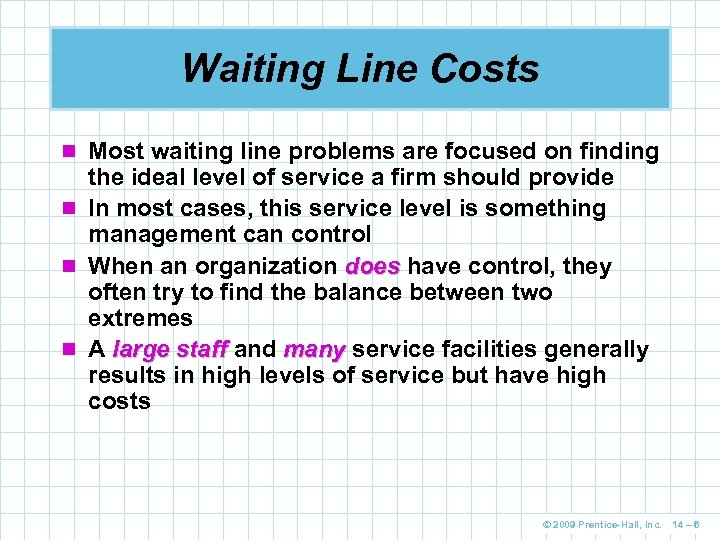Waiting Line Costs n Most waiting line problems are focused on finding the ideal level of service a firm should provide n In most cases, this service level is something management can control n When an organization does have control, they often try to find the balance between two extremes n A large staff and many service facilities generally results in high levels of service but have high costs © 2009 Prentice-Hall, Inc. 14 – 6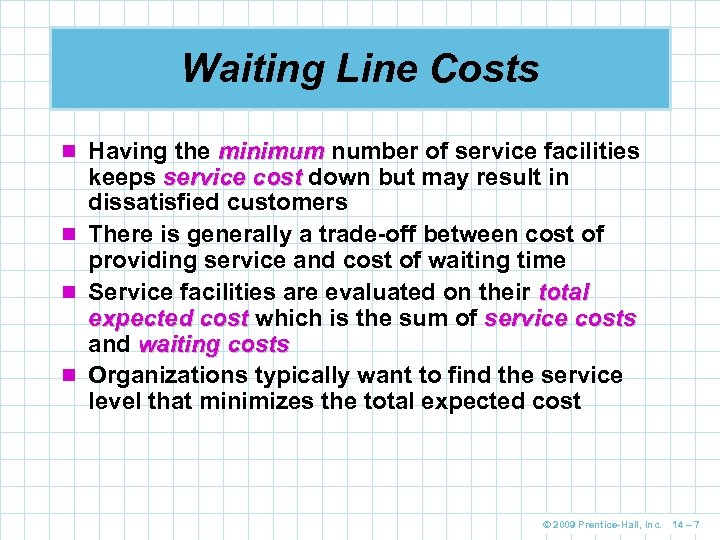Waiting Line Costs n Having the minimum number of service facilities keeps service cost down but may result in dissatisfied customers n There is generally a trade-off between cost of providing service and cost of waiting time n Service facilities are evaluated on their total expected cost which is the sum of service costs and waiting costs n Organizations typically want to find the service level that minimizes the total expected cost © 2009 Prentice-Hall, Inc. 14 – 7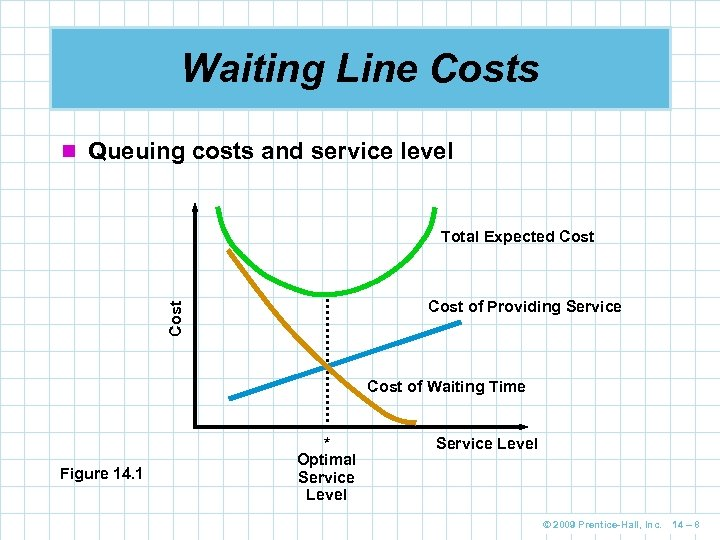Waiting Line Costs n Queuing costs and service level Total Expected Cost of Providing Service Cost of Waiting Time Figure 14. 1 * Optimal Service Level © 2009 Prentice-Hall, Inc. 14 – 8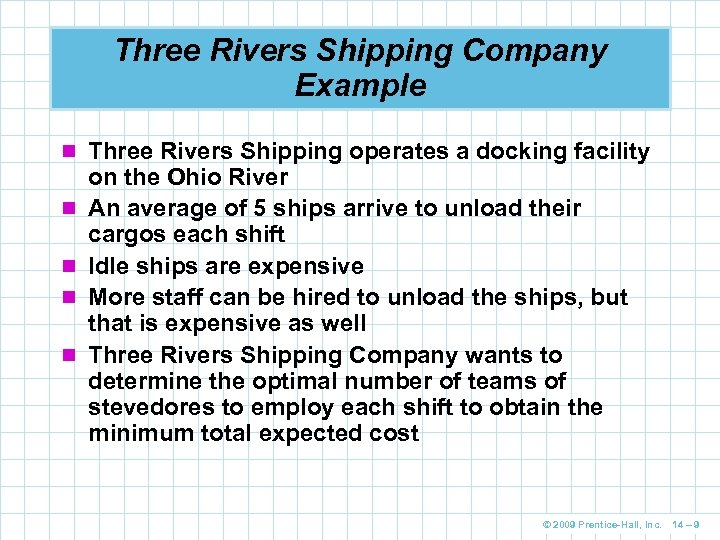Three Rivers Shipping Company Example n Three Rivers Shipping operates a docking facility n n on the Ohio River An average of 5 ships arrive to unload their cargos each shift Idle ships are expensive More staff can be hired to unload the ships, but that is expensive as well Three Rivers Shipping Company wants to determine the optimal number of teams of stevedores to employ each shift to obtain the minimum total expected cost © 2009 Prentice-Hall, Inc. 14 – 9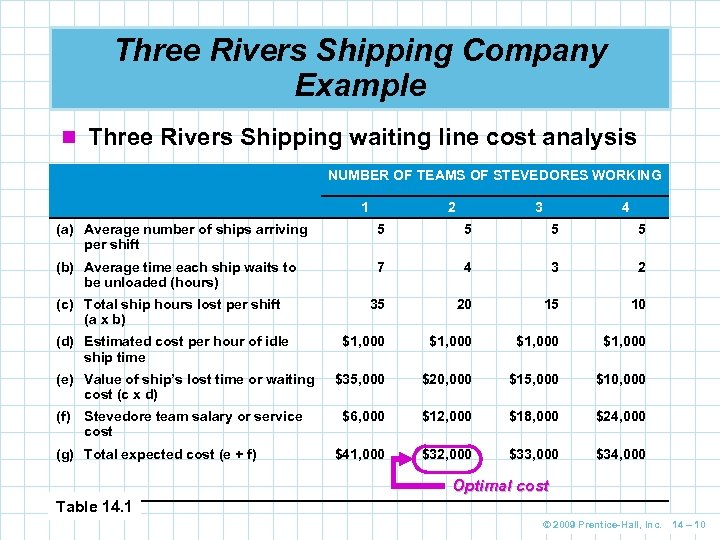Three Rivers Shipping Company Example n Three Rivers Shipping waiting line cost analysis NUMBER OF TEAMS OF STEVEDORES WORKING 1 2 3 4 (a) Average number of ships arriving per shift 5 5 (b) Average time each ship waits to be unloaded (hours) 7 4 3 2 35 20 15 10 \$1, 000 \$35, 000 \$20, 000 \$15, 000 \$10, 000 \$6, 000 \$12, 000 \$18, 000 \$24, 000 \$41, 000 \$32, 000 \$33, 000 \$34, 000 (c) Total ship hours lost per shift (a x b) (d) Estimated cost per hour of idle ship time (e) Value of ship’s lost time or waiting cost (c x d) (f) Stevedore team salary or service cost (g) Total expected cost (e + f) Optimal cost Table 14. 1 © 2009 Prentice-Hall, Inc. 14 – 10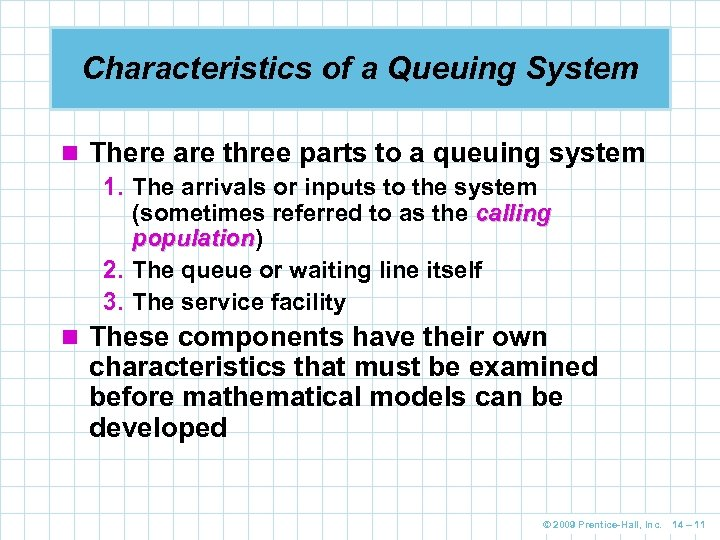Characteristics of a Queuing System n There are three parts to a queuing system 1. The arrivals or inputs to the system (sometimes referred to as the calling population) population 2. The queue or waiting line itself 3. The service facility n These components have their own characteristics that must be examined before mathematical models can be developed © 2009 Prentice-Hall, Inc. 14 – 11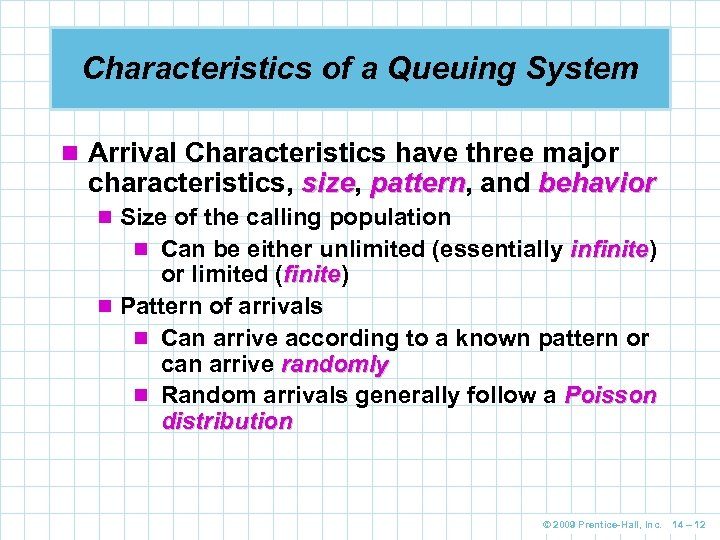Characteristics of a Queuing System n Arrival Characteristics have three major characteristics, size, pattern, and behavior size pattern n Size of the calling population n Can be either unlimited (essentially infinite) infinite or limited (finite) finite n Pattern of arrivals n Can arrive according to a known pattern or can arrive randomly n Random arrivals generally follow a Poisson distribution © 2009 Prentice-Hall, Inc. 14 – 12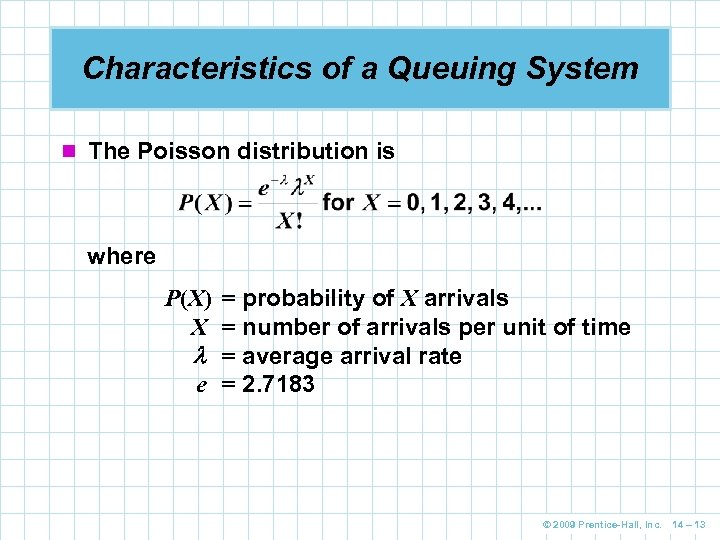Characteristics of a Queuing System n The Poisson distribution is where P(X) = probability of X arrivals X = number of arrivals per unit of time = average arrival rate e = 2. 7183 © 2009 Prentice-Hall, Inc. 14 – 13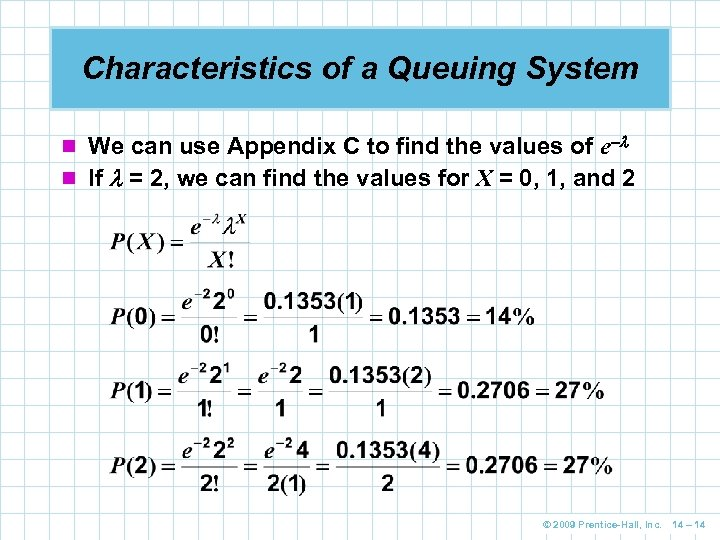Characteristics of a Queuing System n We can use Appendix C to find the values of e– n If = 2, we can find the values for X = 0, 1, and 2 © 2009 Prentice-Hall, Inc. 14 – 14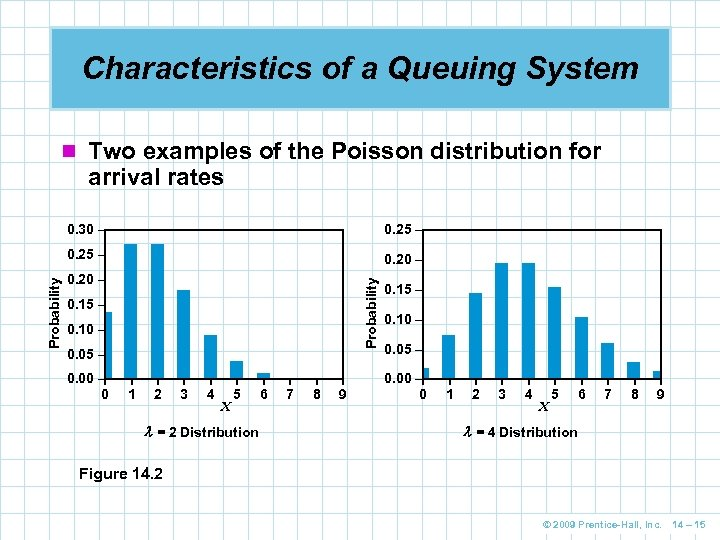Characteristics of a Queuing System n Two examples of the Poisson distribution for arrival rates 0. 25 – 0. 20 – Probability 0. 30 – 0. 15 – 0. 10 – 0. 05 – 0. 00 –| 0 | 1 | 2 | 3 | 4 X | 5 = 2 Distribution | 6 | 7 | 8 | 9 0. 15 – 0. 10 – 0. 05 – 0. 00 –| 0 | 1 | 2 | 3 | 4 X | 5 | 6 | 7 | 8 | 9 = 4 Distribution Figure 14. 2 © 2009 Prentice-Hall, Inc. 14 – 15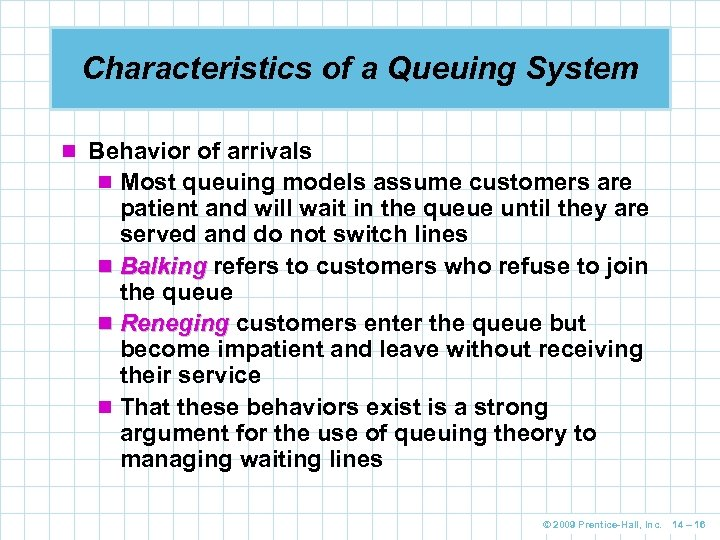Characteristics of a Queuing System n Behavior of arrivals n Most queuing models assume customers are patient and will wait in the queue until they are served and do not switch lines n Balking refers to customers who refuse to join the queue n Reneging customers enter the queue but become impatient and leave without receiving their service n That these behaviors exist is a strong argument for the use of queuing theory to managing waiting lines © 2009 Prentice-Hall, Inc. 14 – 16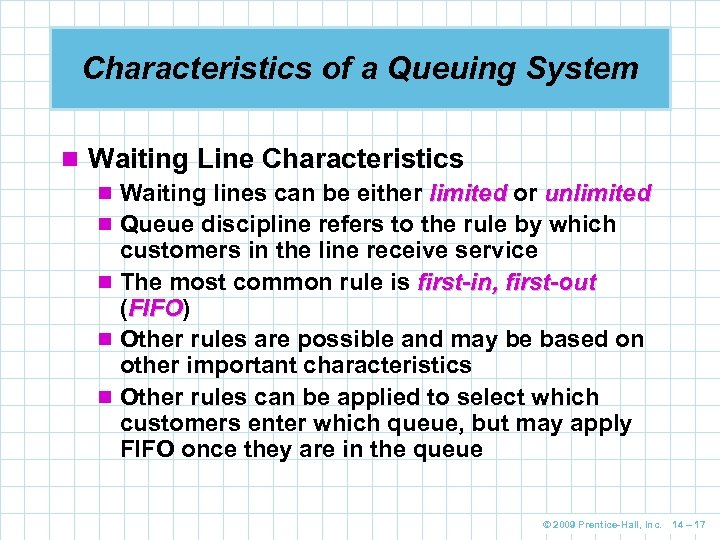Characteristics of a Queuing System n Waiting Line Characteristics n Waiting lines can be either limited or unlimited n Queue discipline refers to the rule by which customers in the line receive service n The most common rule is first-in, first-out (FIFO) FIFO n Other rules are possible and may be based on other important characteristics n Other rules can be applied to select which customers enter which queue, but may apply FIFO once they are in the queue © 2009 Prentice-Hall, Inc. 14 – 17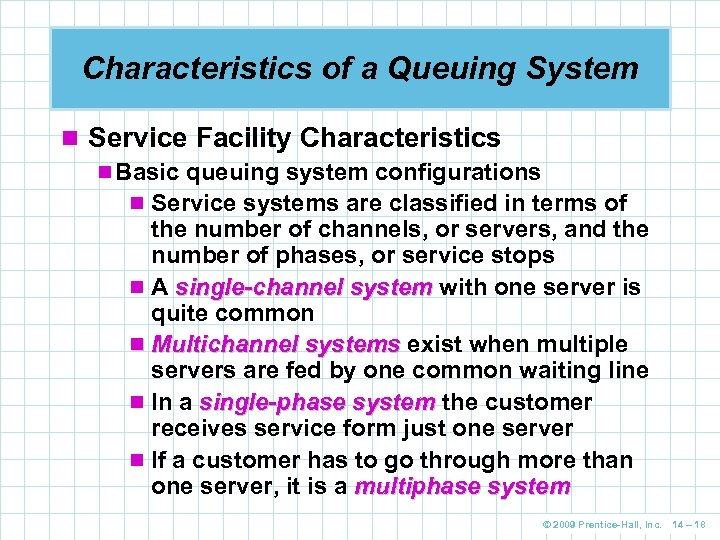Characteristics of a Queuing System n Service Facility Characteristics n Basic queuing system configurations n Service systems are classified in terms of the number of channels, or servers, and the number of phases, or service stops n A single-channel system with one server is quite common n Multichannel systems exist when multiple servers are fed by one common waiting line n In a single-phase system the customer receives service form just one server n If a customer has to go through more than one server, it is a multiphase system © 2009 Prentice-Hall, Inc. 14 – 18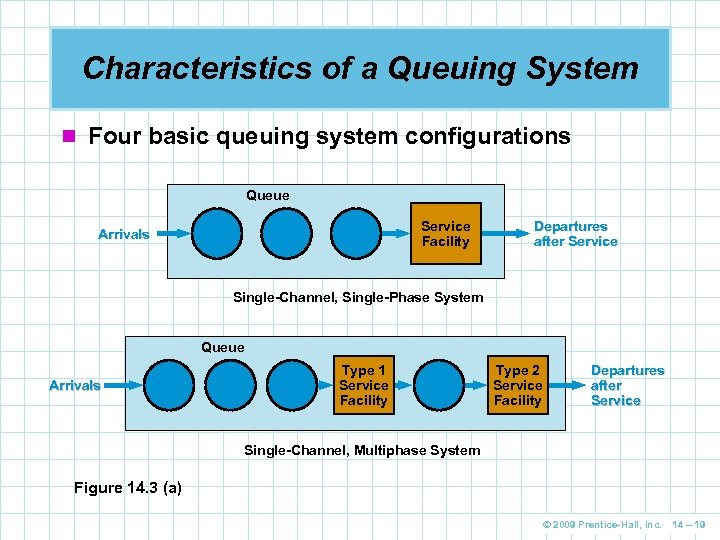Characteristics of a Queuing System n Four basic queuing system configurations Queue Service Facility Arrivals Departures after Service Single-Channel, Single-Phase System Queue Arrivals Type 1 Service Facility Type 2 Service Facility Departures after Service Single-Channel, Multiphase System Figure 14. 3 (a) © 2009 Prentice-Hall, Inc. 14 – 19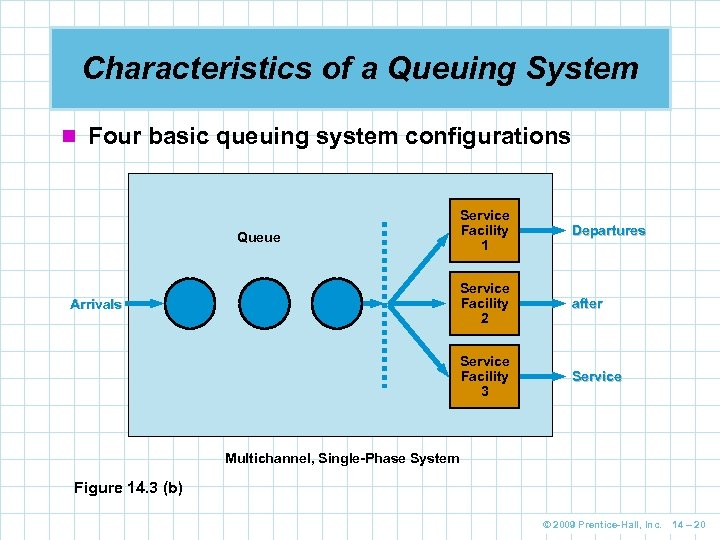Characteristics of a Queuing System n Four basic queuing system configurations after Service Facility 3 Arrivals Departures Service Facility 2 Queue Service Facility 1 Service Multichannel, Single-Phase System Figure 14. 3 (b) © 2009 Prentice-Hall, Inc. 14 – 20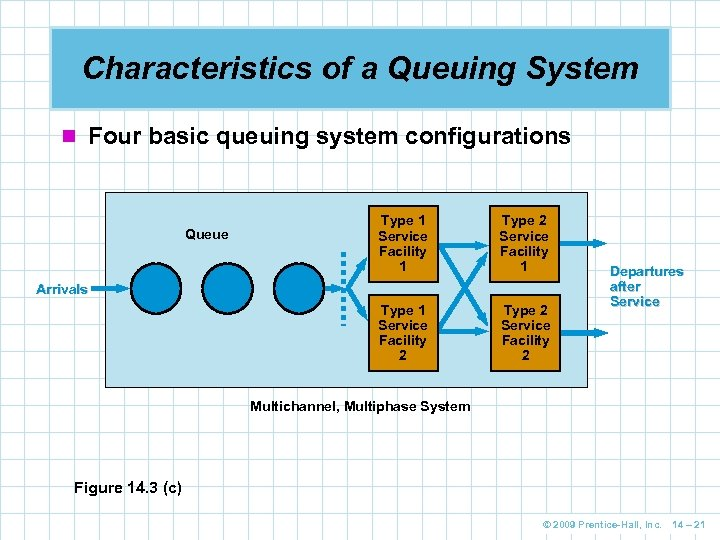Characteristics of a Queuing System n Four basic queuing system configurations Type 1 Service Facility 1 Type 2 Service Facility 1 Type 1 Service Facility 2 Queue Type 2 Service Facility 2 Arrivals Departures after Service Multichannel, Multiphase System Figure 14. 3 (c) © 2009 Prentice-Hall, Inc. 14 – 21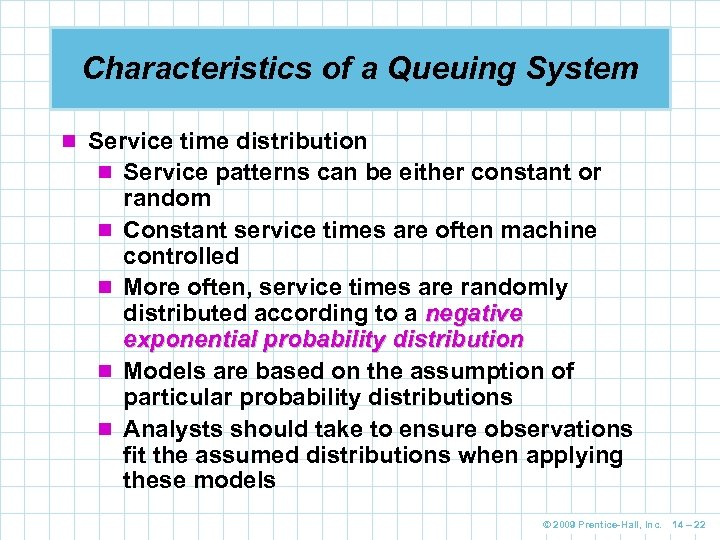Characteristics of a Queuing System n Service time distribution n Service patterns can be either constant or n n random Constant service times are often machine controlled More often, service times are randomly distributed according to a negative exponential probability distribution Models are based on the assumption of particular probability distributions Analysts should take to ensure observations fit the assumed distributions when applying these models © 2009 Prentice-Hall, Inc. 14 – 22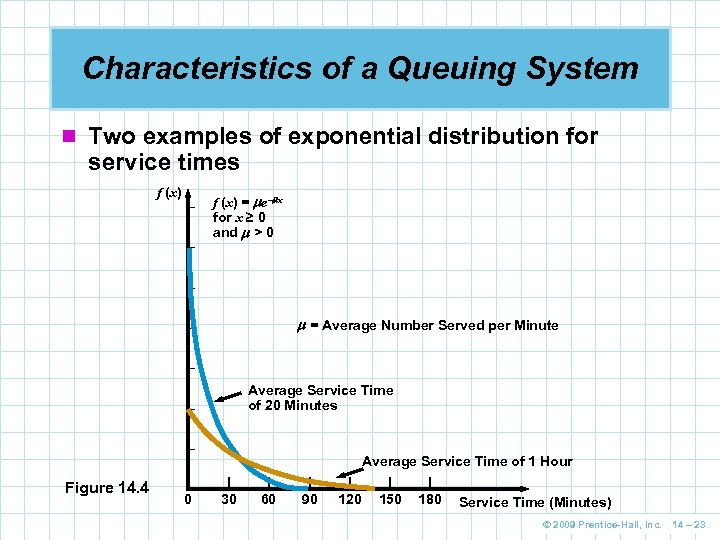Characteristics of a Queuing System n Two examples of exponential distribution for service times f (x) – – f (x) = e– x for x ≥ 0 and > 0 – = Average Number Served per Minute – – Average Service Time of 20 Minutes – – Figure 14. 4 |– 0 | 30 | 60 | 90 Average Service Time of 1 Hour | | | 120 150 180 Service Time (Minutes) © 2009 Prentice-Hall, Inc. 14 – 23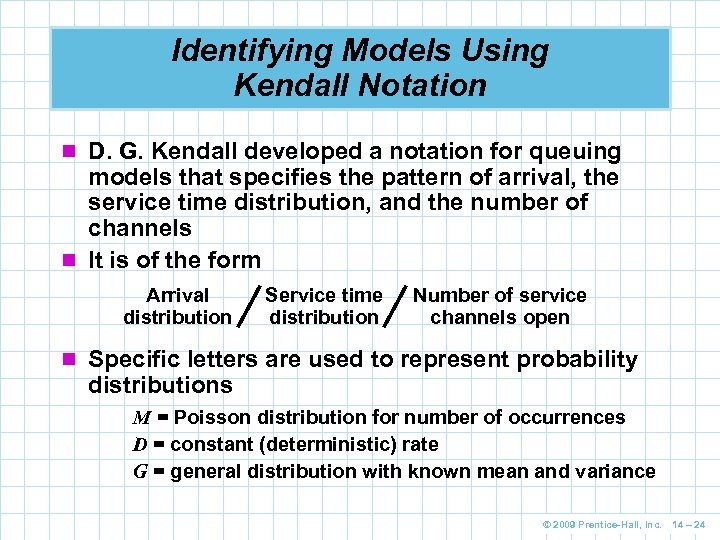Identifying Models Using Kendall Notation n D. G. Kendall developed a notation for queuing models that specifies the pattern of arrival, the service time distribution, and the number of channels n It is of the form Arrival distribution Service time distribution Number of service channels open n Specific letters are used to represent probability distributions M = Poisson distribution for number of occurrences D = constant (deterministic) rate G = general distribution with known mean and variance © 2009 Prentice-Hall, Inc. 14 – 24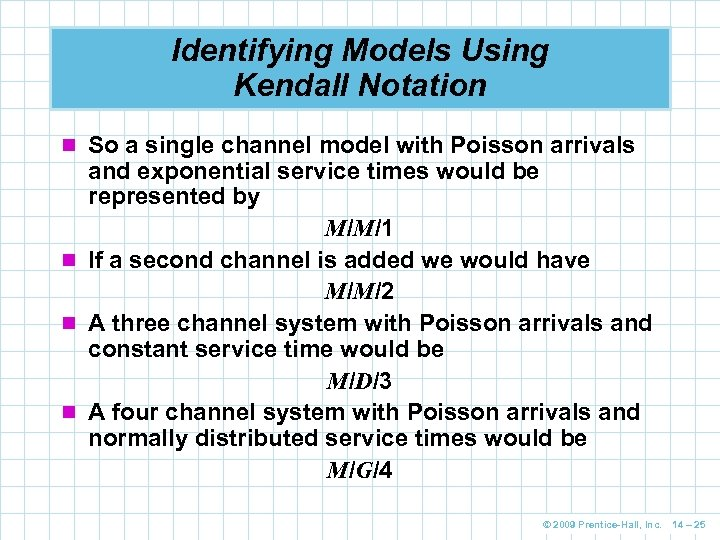Identifying Models Using Kendall Notation n So a single channel model with Poisson arrivals and exponential service times would be represented by M/M/1 n If a second channel is added we would have M/M/2 n A three channel system with Poisson arrivals and constant service time would be M/D/3 n A four channel system with Poisson arrivals and normally distributed service times would be M/G/4 © 2009 Prentice-Hall, Inc. 14 – 25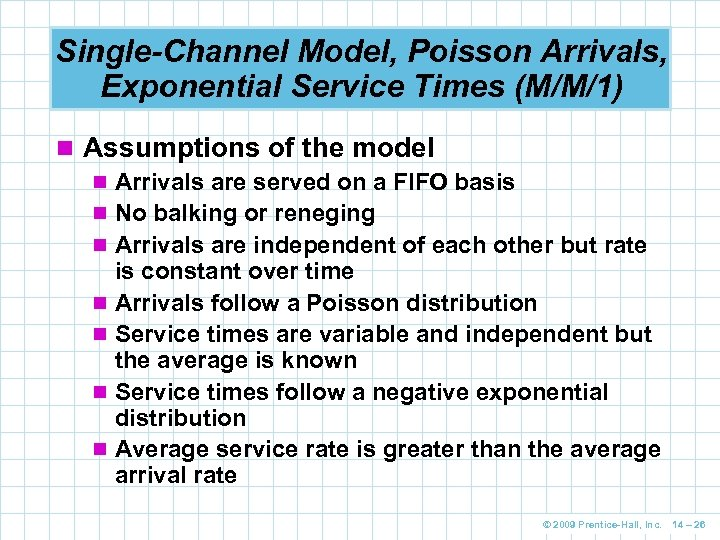Single-Channel Model, Poisson Arrivals, Exponential Service Times (M/M/1) n Assumptions of the model n Arrivals are served on a FIFO basis n No balking or reneging n Arrivals are independent of each other but rate is constant over time n Arrivals follow a Poisson distribution n Service times are variable and independent but the average is known n Service times follow a negative exponential distribution n Average service rate is greater than the average arrival rate © 2009 Prentice-Hall, Inc. 14 – 26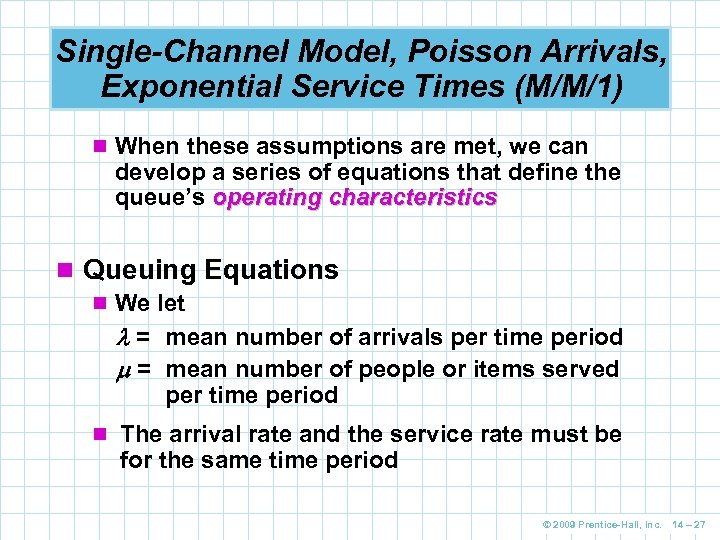Single-Channel Model, Poisson Arrivals, Exponential Service Times (M/M/1) n When these assumptions are met, we can develop a series of equations that define the queue’s operating characteristics n Queuing Equations n We let = mean number of arrivals per time period = mean number of people or items served per time period n The arrival rate and the service rate must be for the same time period © 2009 Prentice-Hall, Inc. 14 – 27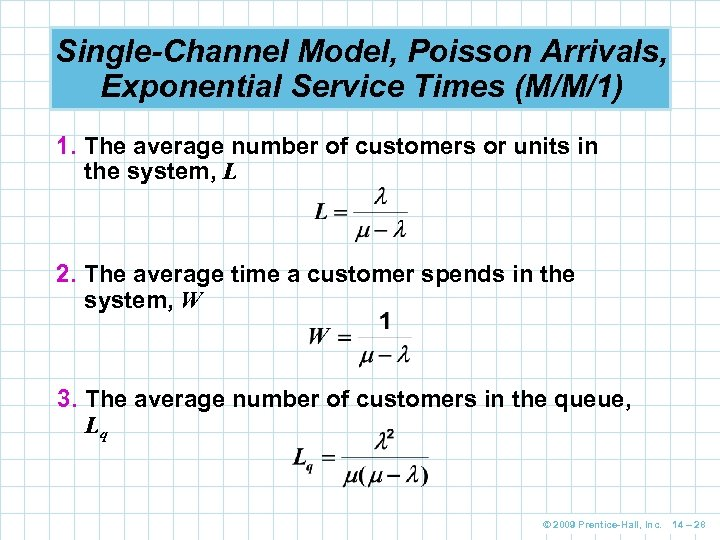Single-Channel Model, Poisson Arrivals, Exponential Service Times (M/M/1) 1. The average number of customers or units in the system, L 2. The average time a customer spends in the system, W 3. The average number of customers in the queue, Lq © 2009 Prentice-Hall, Inc. 14 – 28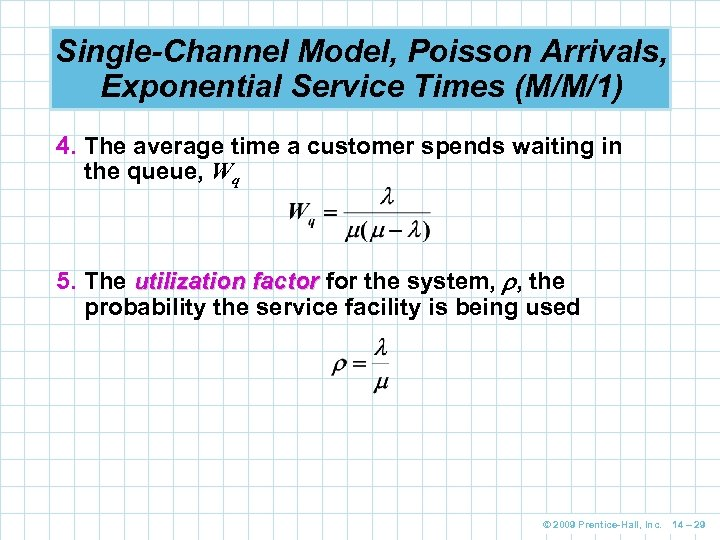Single-Channel Model, Poisson Arrivals, Exponential Service Times (M/M/1) 4. The average time a customer spends waiting in the queue, Wq 5. The utilization factor for the system, , the probability the service facility is being used © 2009 Prentice-Hall, Inc. 14 – 29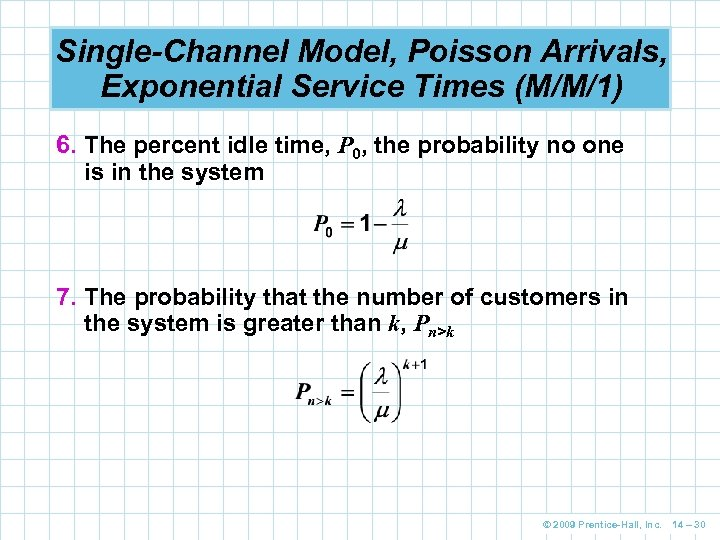Single-Channel Model, Poisson Arrivals, Exponential Service Times (M/M/1) 6. The percent idle time, P 0, the probability no one is in the system 7. The probability that the number of customers in the system is greater than k, Pn>k © 2009 Prentice-Hall, Inc. 14 – 30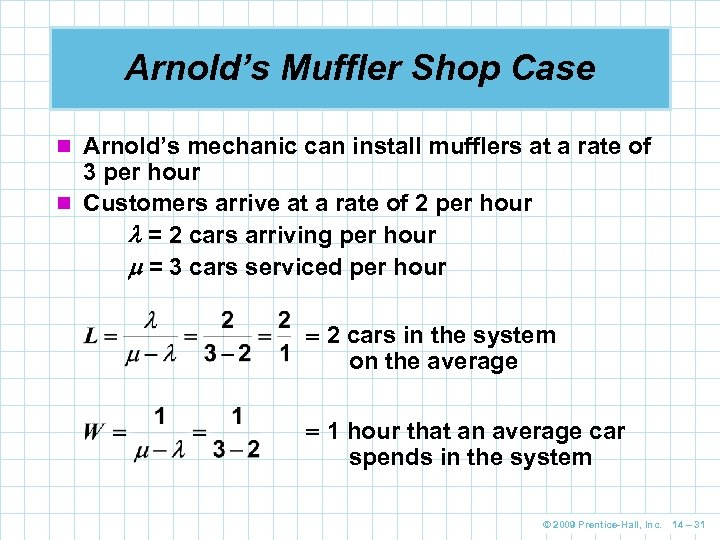Arnold’s Muffler Shop Case n Arnold’s mechanic can install mufflers at a rate of 3 per hour n Customers arrive at a rate of 2 per hour = 2 cars arriving per hour = 3 cars serviced per hour 2 cars in the system on the average 1 hour that an average car spends in the system © 2009 Prentice-Hall, Inc. 14 – 31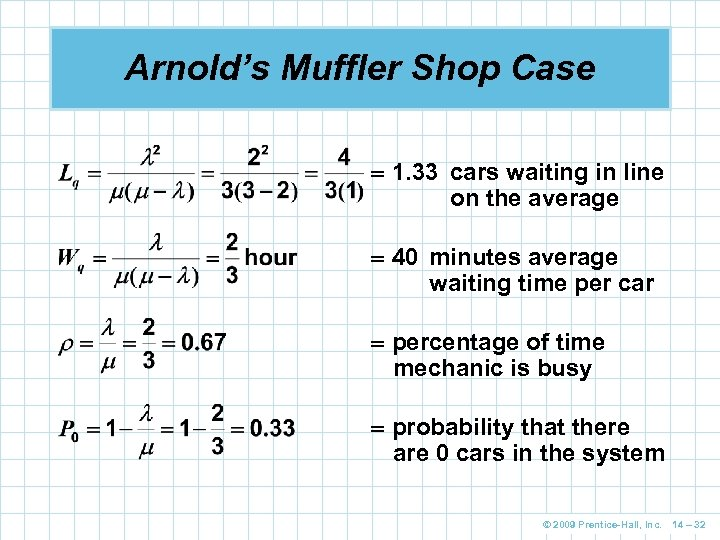Arnold’s Muffler Shop Case 1. 33 cars waiting in line on the average 40 minutes average waiting time per car percentage of time mechanic is busy probability that there are 0 cars in the system © 2009 Prentice-Hall, Inc. 14 – 32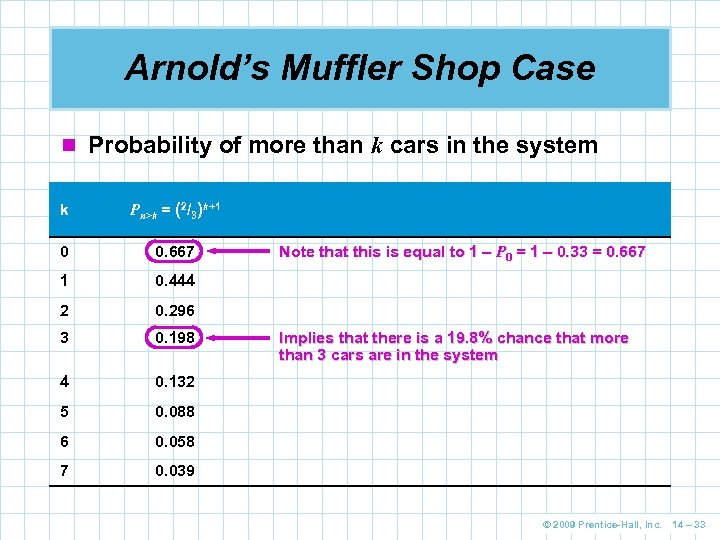Arnold’s Muffler Shop Case n Probability of more than k cars in the system k Pn>k = (2/3)k+1 0 0. 667 1 0. 444 2 0. 296 3 0. 198 4 0. 132 5 0. 088 6 0. 058 7 0. 039 Note that this is equal to 1 – P 0 = 1 – 0. 33 = 0. 667 Implies that there is a 19. 8% chance that more than 3 cars are in the system © 2009 Prentice-Hall, Inc. 14 – 33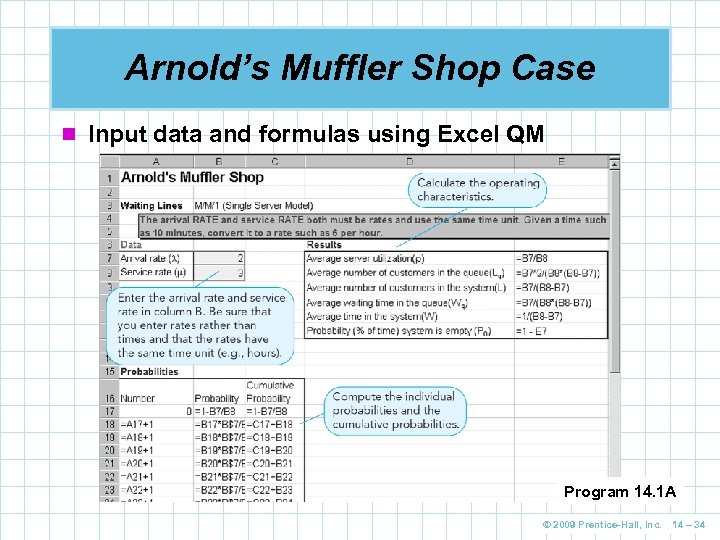Arnold’s Muffler Shop Case n Input data and formulas using Excel QM Program 14. 1 A © 2009 Prentice-Hall, Inc. 14 – 34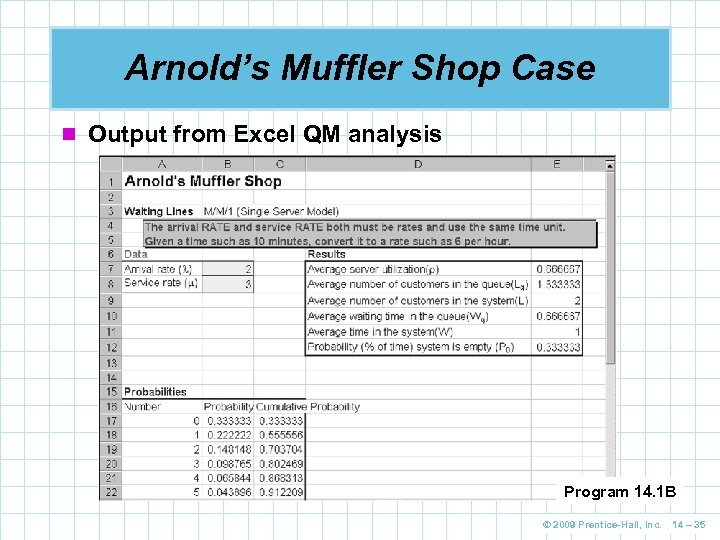Arnold’s Muffler Shop Case n Output from Excel QM analysis Program 14. 1 B © 2009 Prentice-Hall, Inc. 14 – 35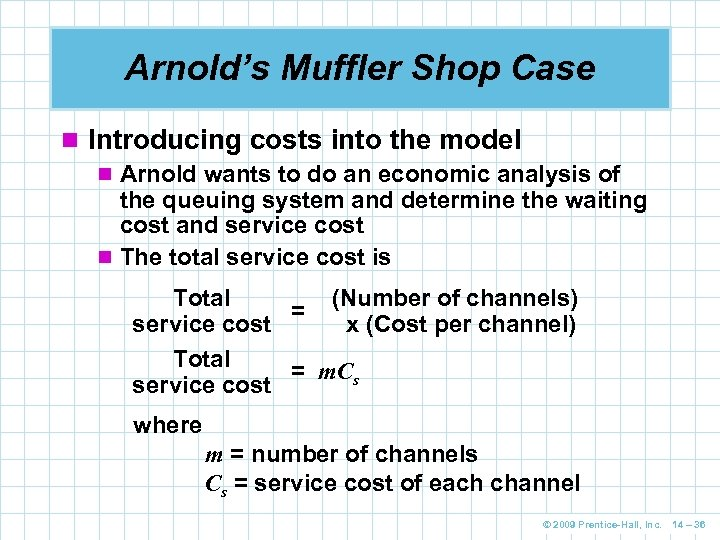Arnold’s Muffler Shop Case n Introducing costs into the model n Arnold wants to do an economic analysis of the queuing system and determine the waiting cost and service cost n The total service cost is Total (Number of channels) = service cost x (Cost per channel) Total = m. Cs service cost where m = number of channels Cs = service cost of each channel © 2009 Prentice-Hall, Inc. 14 – 36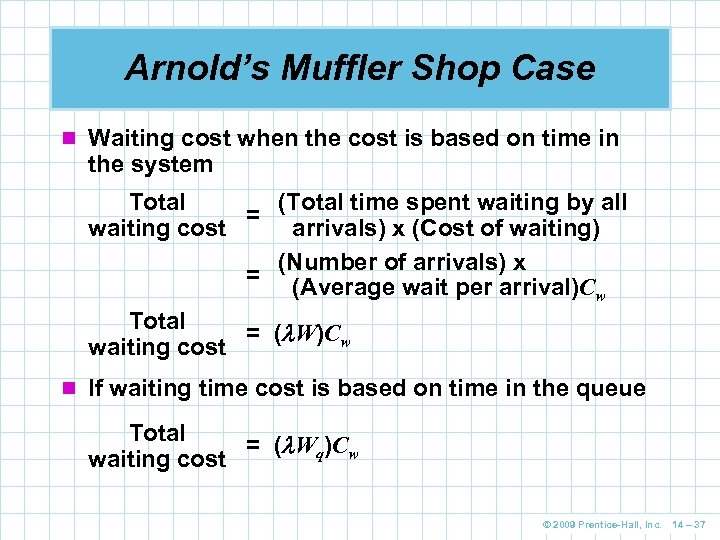Arnold’s Muffler Shop Case n Waiting cost when the cost is based on time in the system Total (Total time spent waiting by all = waiting cost arrivals) x (Cost of waiting) (Number of arrivals) x = (Average wait per arrival)Cw Total = ( W)Cw waiting cost n If waiting time cost is based on time in the queue Total = ( Wq)Cw waiting cost © 2009 Prentice-Hall, Inc. 14 – 37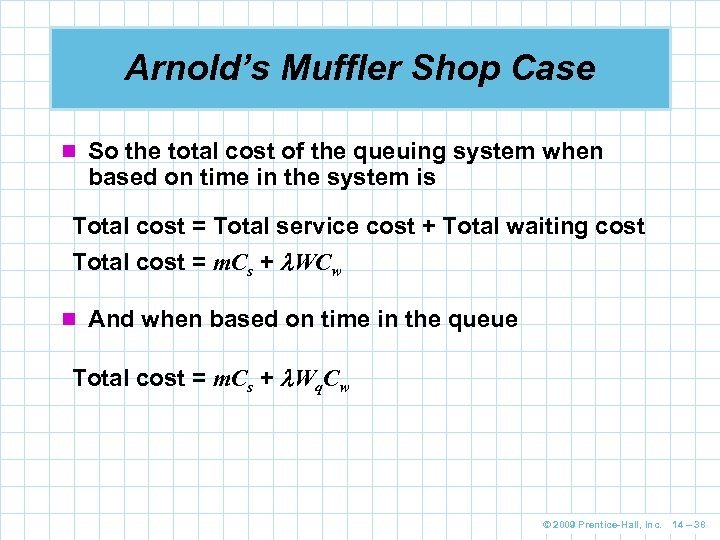Arnold’s Muffler Shop Case n So the total cost of the queuing system when based on time in the system is Total cost = Total service cost + Total waiting cost Total cost = m. Cs + WCw n And when based on time in the queue Total cost = m. Cs + Wq. Cw © 2009 Prentice-Hall, Inc. 14 – 38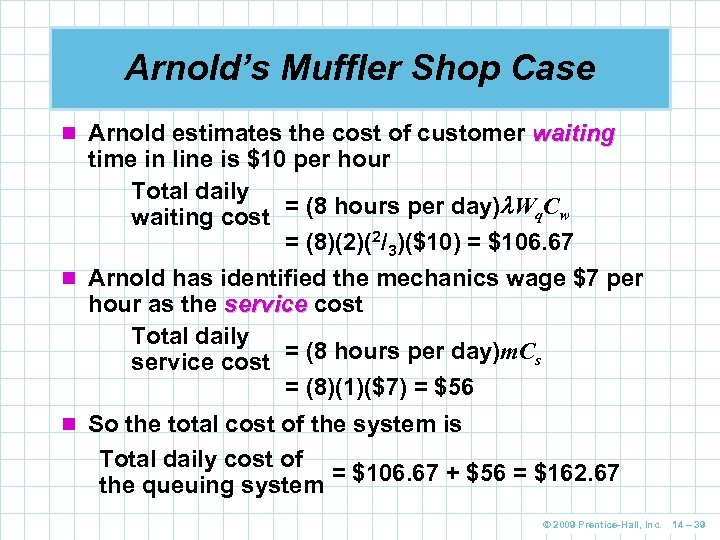Arnold’s Muffler Shop Case n Arnold estimates the cost of customer waiting time in line is \$10 per hour Total daily waiting cost = (8 hours per day) Wq. Cw = (8)(2)(2/3)(\$10) = \$106. 67 n Arnold has identified the mechanics wage \$7 per hour as the service cost Total daily service cost = (8 hours per day)m. Cs = (8)(1)(\$7) = \$56 n So the total cost of the system is Total daily cost of the queuing system = \$106. 67 + \$56 = \$162. 67 © 2009 Prentice-Hall, Inc. 14 – 39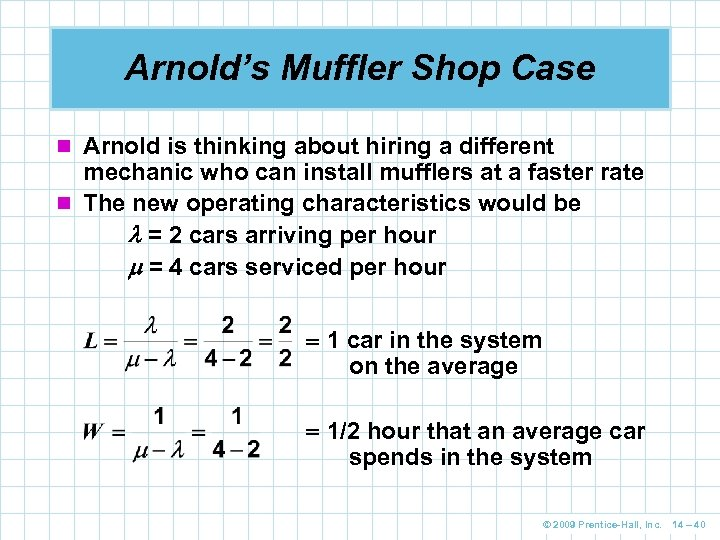Arnold’s Muffler Shop Case n Arnold is thinking about hiring a different mechanic who can install mufflers at a faster rate n The new operating characteristics would be = 2 cars arriving per hour = 4 cars serviced per hour 1 car in the system on the average 1/2 hour that an average car spends in the system © 2009 Prentice-Hall, Inc. 14 – 40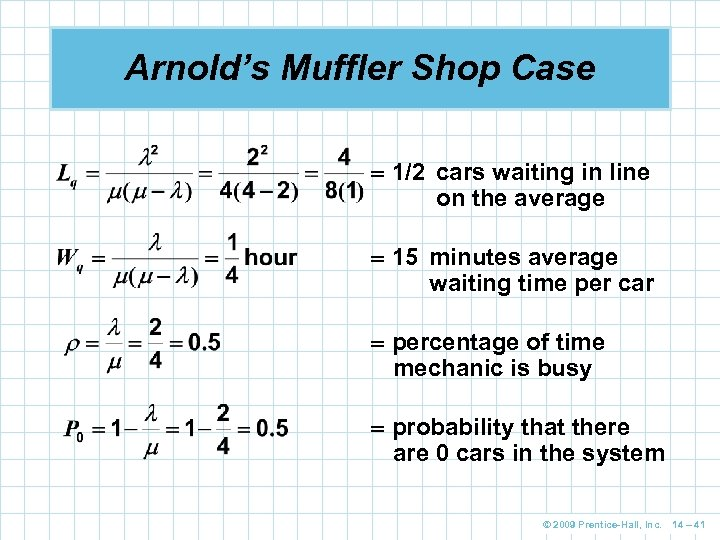Arnold’s Muffler Shop Case 1/2 cars waiting in line on the average 15 minutes average waiting time per car percentage of time mechanic is busy probability that there are 0 cars in the system © 2009 Prentice-Hall, Inc. 14 – 41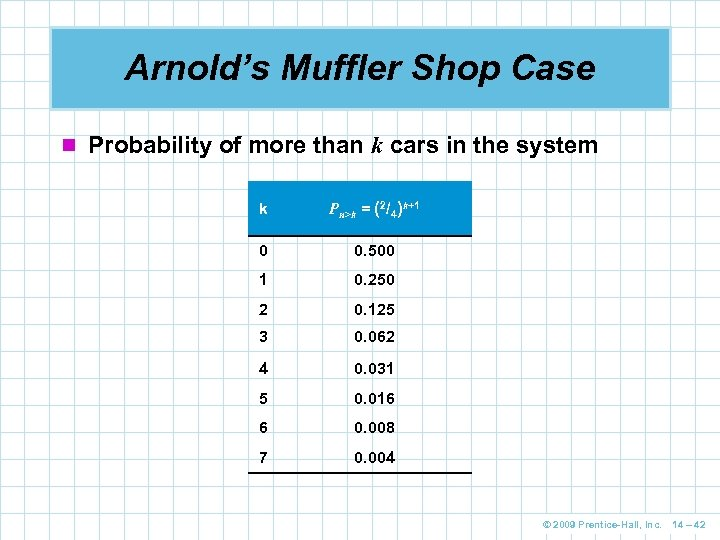Arnold’s Muffler Shop Case n Probability of more than k cars in the system k Pn>k = (2/4)k+1 0 0. 500 1 0. 250 2 0. 125 3 0. 062 4 0. 031 5 0. 016 6 0. 008 7 0. 004 © 2009 Prentice-Hall, Inc. 14 – 42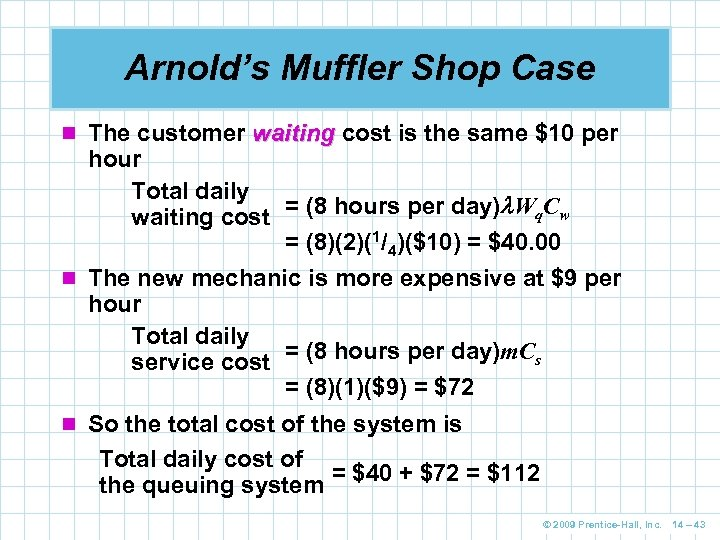Arnold’s Muffler Shop Case n The customer waiting cost is the same \$10 per hour Total daily waiting cost = (8 hours per day) Wq. Cw = (8)(2)(1/4)(\$10) = \$40. 00 n The new mechanic is more expensive at \$9 per hour Total daily service cost = (8 hours per day)m. Cs = (8)(1)(\$9) = \$72 n So the total cost of the system is Total daily cost of the queuing system = \$40 + \$72 = \$112 © 2009 Prentice-Hall, Inc. 14 – 43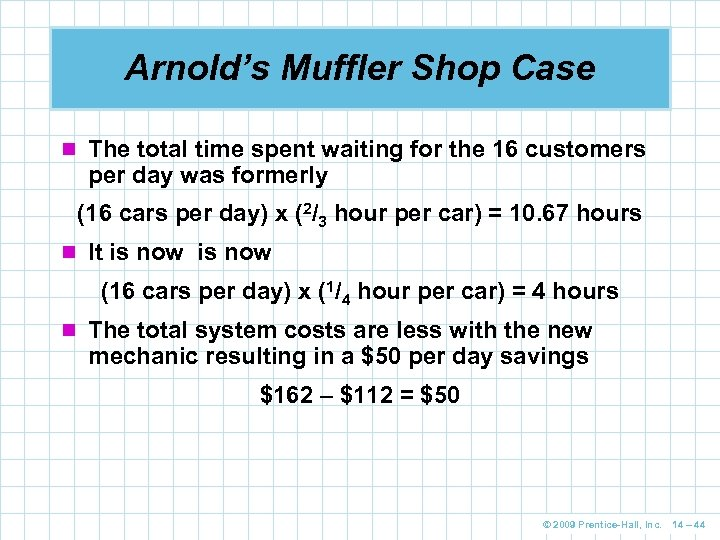Arnold’s Muffler Shop Case n The total time spent waiting for the 16 customers per day was formerly (16 cars per day) x (2/3 hour per car) = 10. 67 hours n It is now (16 cars per day) x (1/4 hour per car) = 4 hours n The total system costs are less with the new mechanic resulting in a \$50 per day savings \$162 – \$112 = \$50 © 2009 Prentice-Hall, Inc. 14 – 44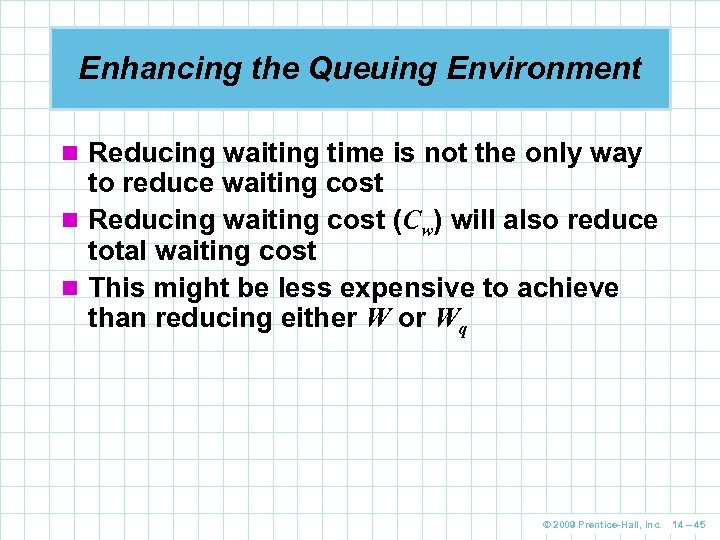Enhancing the Queuing Environment n Reducing waiting time is not the only way to reduce waiting cost n Reducing waiting cost (Cw) will also reduce total waiting cost n This might be less expensive to achieve than reducing either W or Wq © 2009 Prentice-Hall, Inc. 14 – 45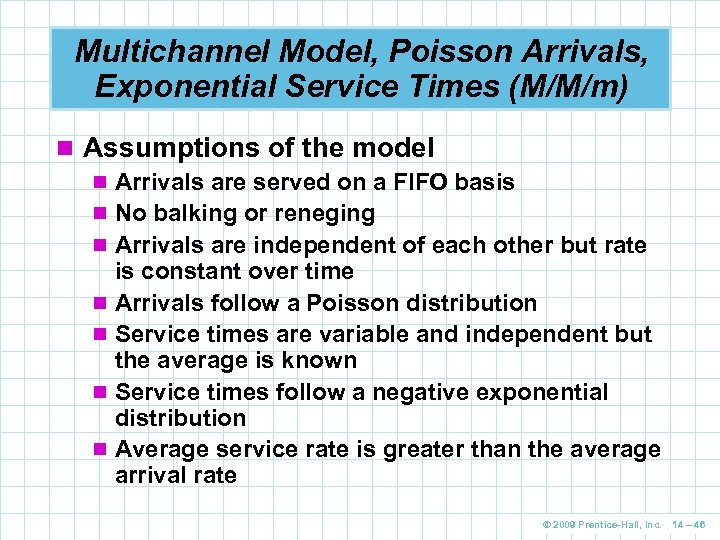Multichannel Model, Poisson Arrivals, Exponential Service Times (M/M/m) n Assumptions of the model n Arrivals are served on a FIFO basis n No balking or reneging n Arrivals are independent of each other but rate is constant over time n Arrivals follow a Poisson distribution n Service times are variable and independent but the average is known n Service times follow a negative exponential distribution n Average service rate is greater than the average arrival rate © 2009 Prentice-Hall, Inc. 14 – 46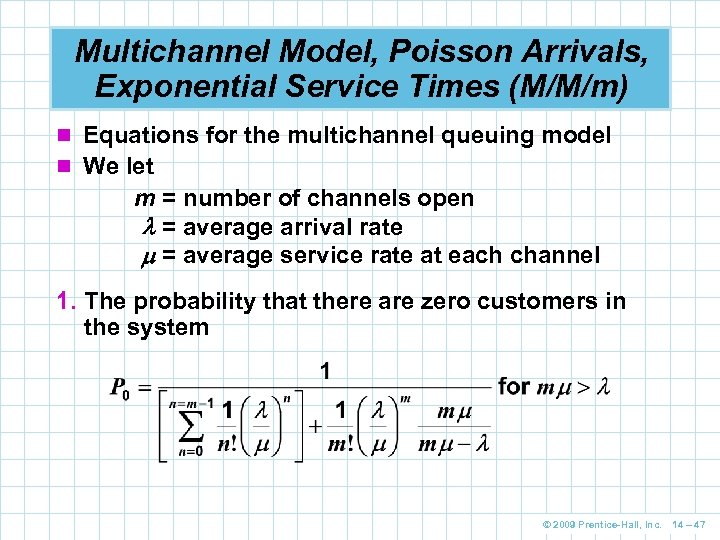Multichannel Model, Poisson Arrivals, Exponential Service Times (M/M/m) n Equations for the multichannel queuing model n We let m = number of channels open = average arrival rate = average service rate at each channel 1. The probability that there are zero customers in the system © 2009 Prentice-Hall, Inc. 14 – 47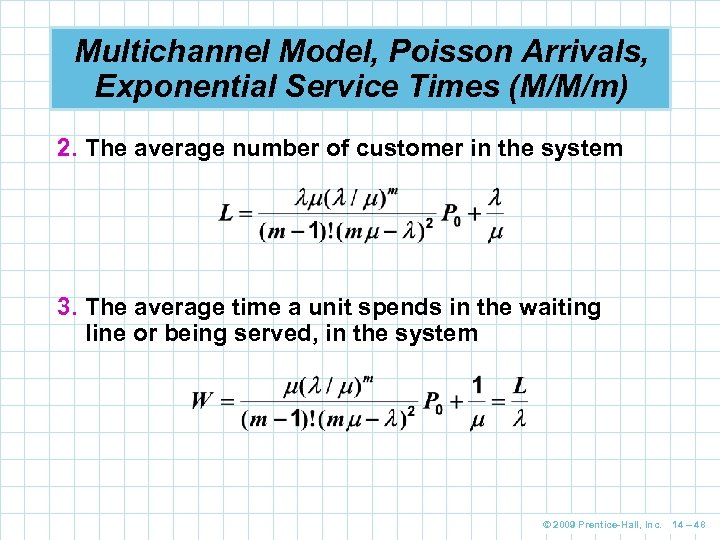Multichannel Model, Poisson Arrivals, Exponential Service Times (M/M/m) 2. The average number of customer in the system 3. The average time a unit spends in the waiting line or being served, in the system © 2009 Prentice-Hall, Inc. 14 – 48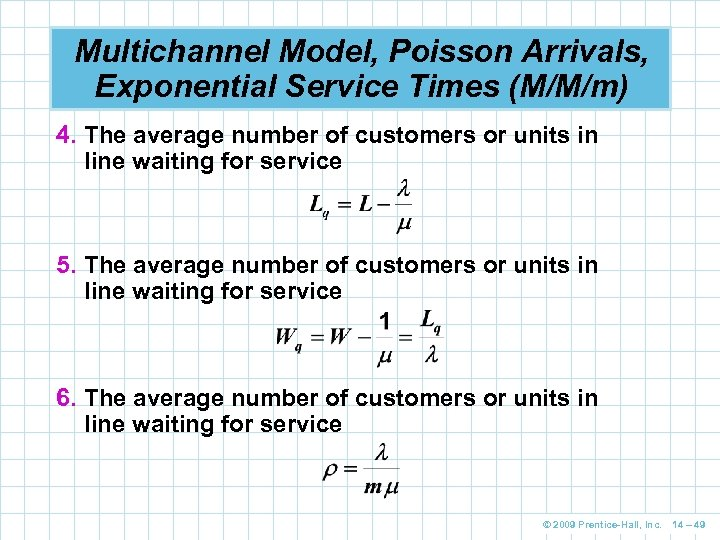Multichannel Model, Poisson Arrivals, Exponential Service Times (M/M/m) 4. The average number of customers or units in line waiting for service 5. The average number of customers or units in line waiting for service 6. The average number of customers or units in line waiting for service © 2009 Prentice-Hall, Inc. 14 – 49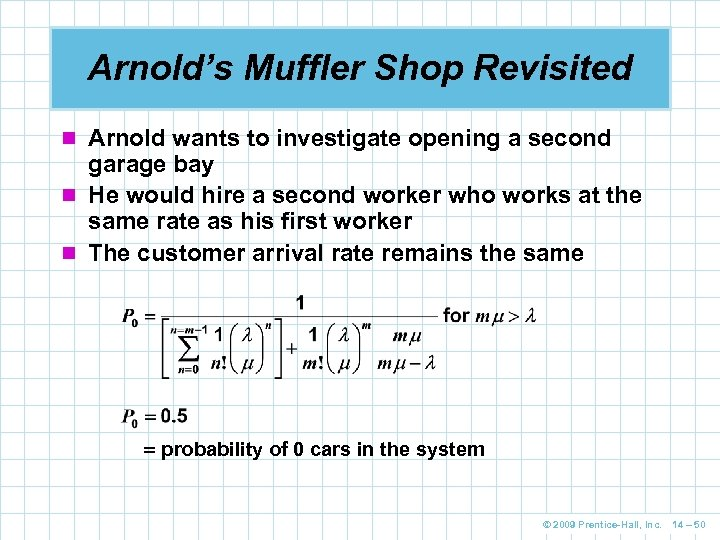Arnold’s Muffler Shop Revisited n Arnold wants to investigate opening a second garage bay n He would hire a second worker who works at the same rate as his first worker n The customer arrival rate remains the same probability of 0 cars in the system © 2009 Prentice-Hall, Inc. 14 – 50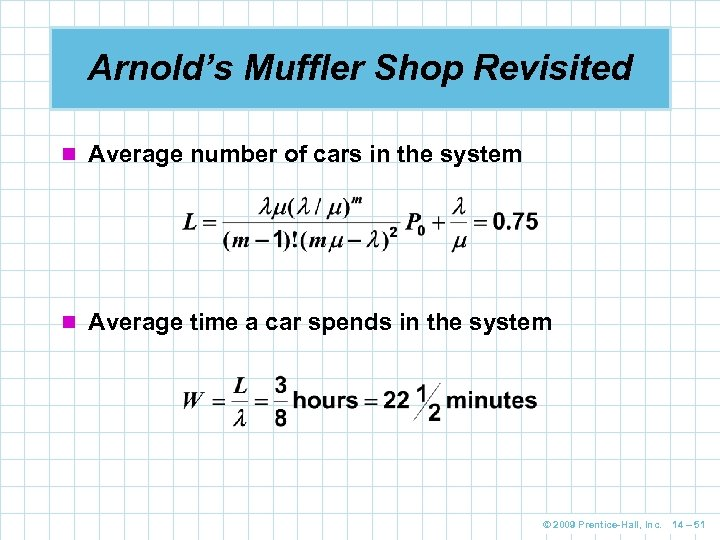Arnold’s Muffler Shop Revisited n Average number of cars in the system n Average time a car spends in the system © 2009 Prentice-Hall, Inc. 14 – 51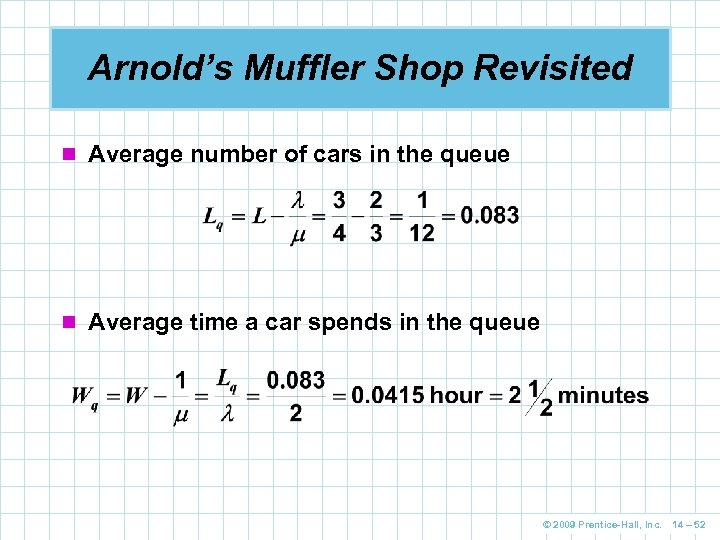Arnold’s Muffler Shop Revisited n Average number of cars in the queue n Average time a car spends in the queue © 2009 Prentice-Hall, Inc. 14 – 52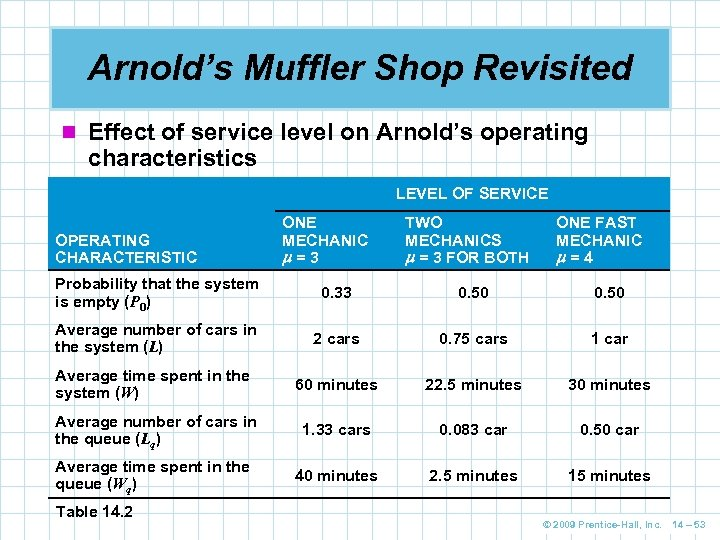Arnold’s Muffler Shop Revisited n Effect of service level on Arnold’s operating characteristics LEVEL OF SERVICE OPERATING CHARACTERISTIC ONE MECHANIC =3 TWO MECHANICS = 3 FOR BOTH ONE FAST MECHANIC =4 Probability that the system is empty (P 0) 0. 33 0. 50 Average number of cars in the system (L) 2 cars 0. 75 cars 1 car Average time spent in the system (W) 60 minutes 22. 5 minutes 30 minutes Average number of cars in the queue (Lq) 1. 33 cars 0. 083 car 0. 50 car Average time spent in the queue (Wq) 40 minutes 2. 5 minutes 15 minutes Table 14. 2 © 2009 Prentice-Hall, Inc. 14 – 53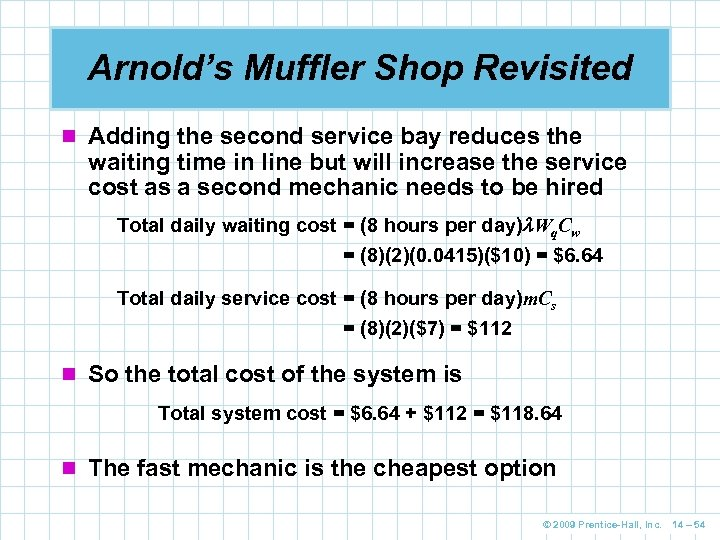Arnold’s Muffler Shop Revisited n Adding the second service bay reduces the waiting time in line but will increase the service cost as a second mechanic needs to be hired Total daily waiting cost = (8 hours per day) Wq. Cw = (8)(2)(0. 0415)(\$10) = \$6. 64 Total daily service cost = (8 hours per day)m. Cs = (8)(2)(\$7) = \$112 n So the total cost of the system is Total system cost = \$6. 64 + \$112 = \$118. 64 n The fast mechanic is the cheapest option © 2009 Prentice-Hall, Inc. 14 – 54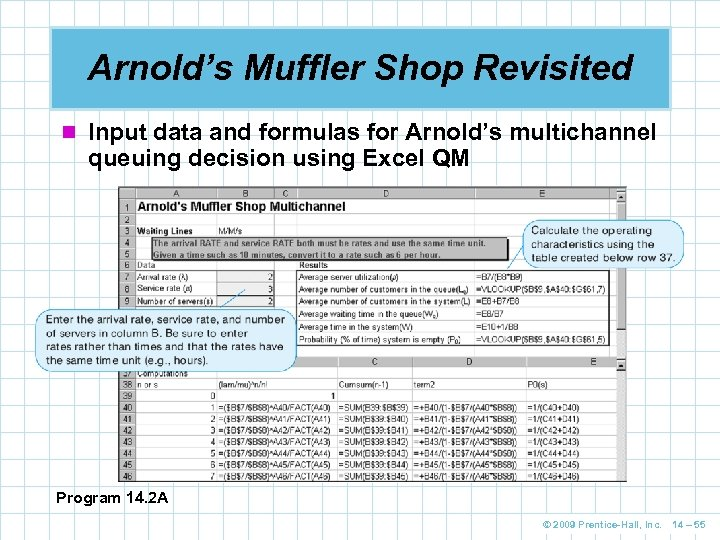Arnold’s Muffler Shop Revisited n Input data and formulas for Arnold’s multichannel queuing decision using Excel QM Program 14. 2 A © 2009 Prentice-Hall, Inc. 14 – 55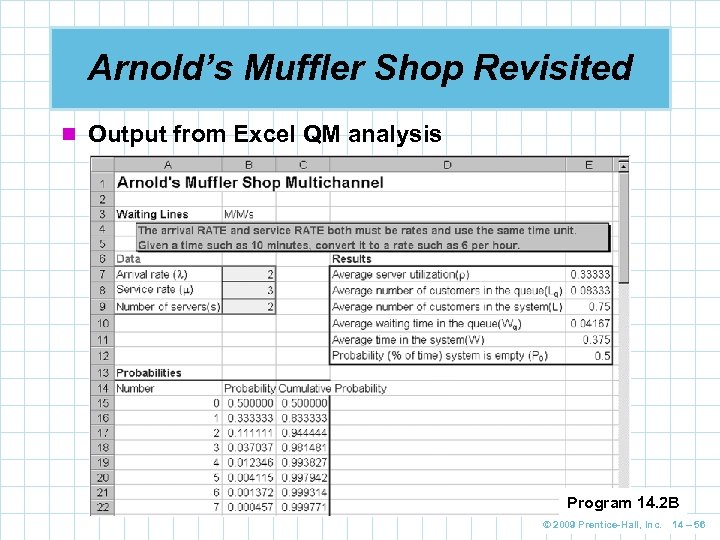Arnold’s Muffler Shop Revisited n Output from Excel QM analysis Program 14. 2 B © 2009 Prentice-Hall, Inc. 14 – 56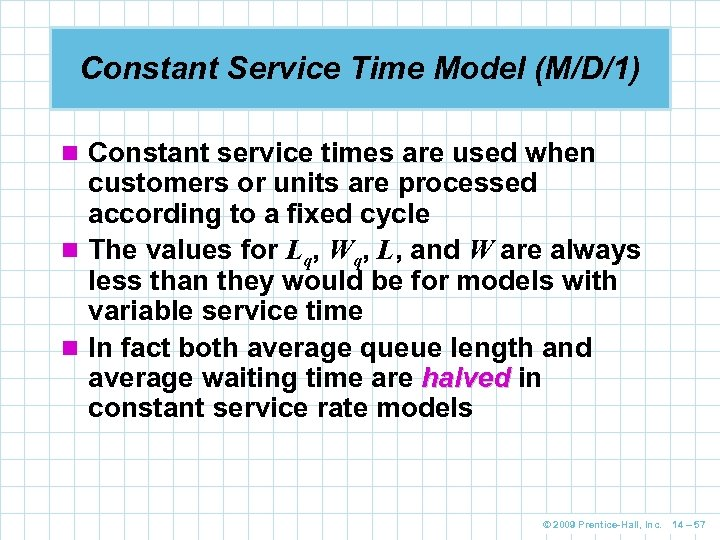Constant Service Time Model (M/D/1) n Constant service times are used when customers or units are processed according to a fixed cycle n The values for Lq, Wq, L, and W are always less than they would be for models with variable service time n In fact both average queue length and average waiting time are halved in constant service rate models © 2009 Prentice-Hall, Inc. 14 – 57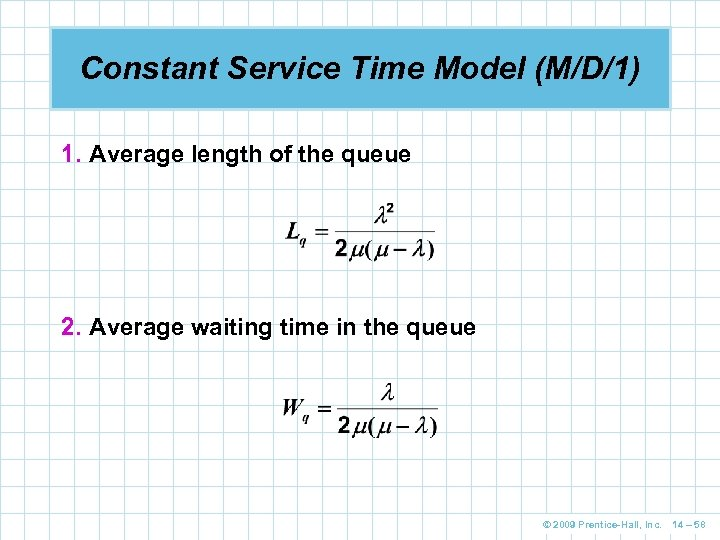Constant Service Time Model (M/D/1) 1. Average length of the queue 2. Average waiting time in the queue © 2009 Prentice-Hall, Inc. 14 – 58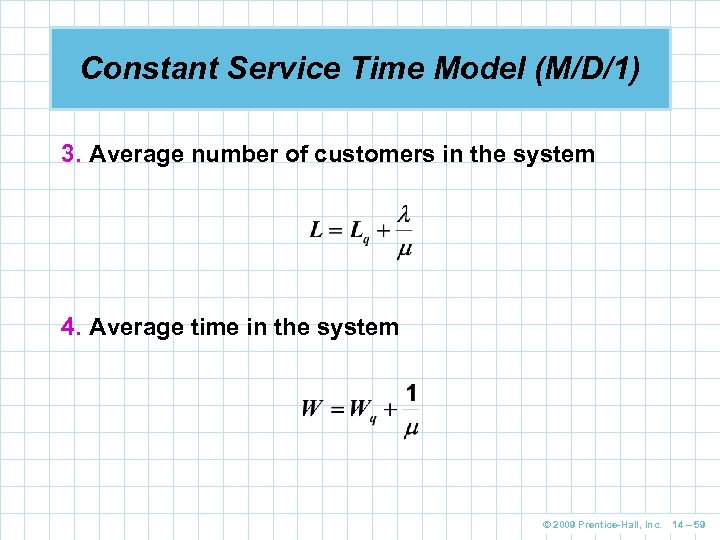Constant Service Time Model (M/D/1) 3. Average number of customers in the system 4. Average time in the system © 2009 Prentice-Hall, Inc. 14 – 59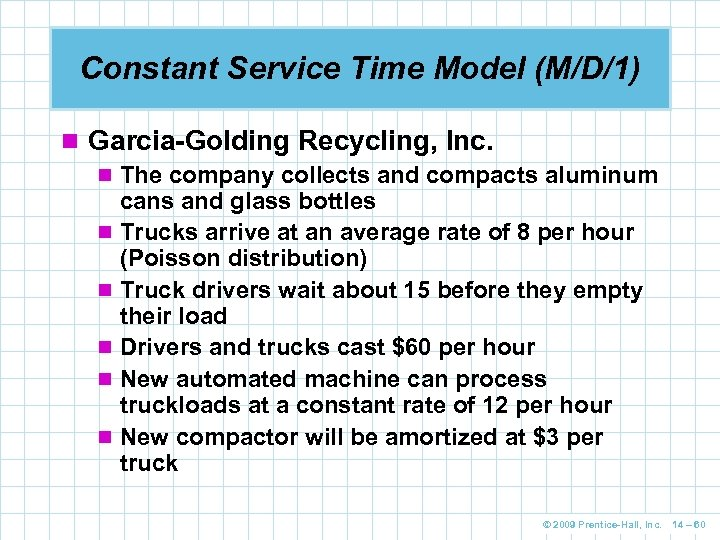Constant Service Time Model (M/D/1) n Garcia-Golding Recycling, Inc. n The company collects and compacts aluminum cans and glass bottles n Trucks arrive at an average rate of 8 per hour (Poisson distribution) n Truck drivers wait about 15 before they empty their load n Drivers and trucks cast \$60 per hour n New automated machine can process truckloads at a constant rate of 12 per hour n New compactor will be amortized at \$3 per truck © 2009 Prentice-Hall, Inc. 14 – 60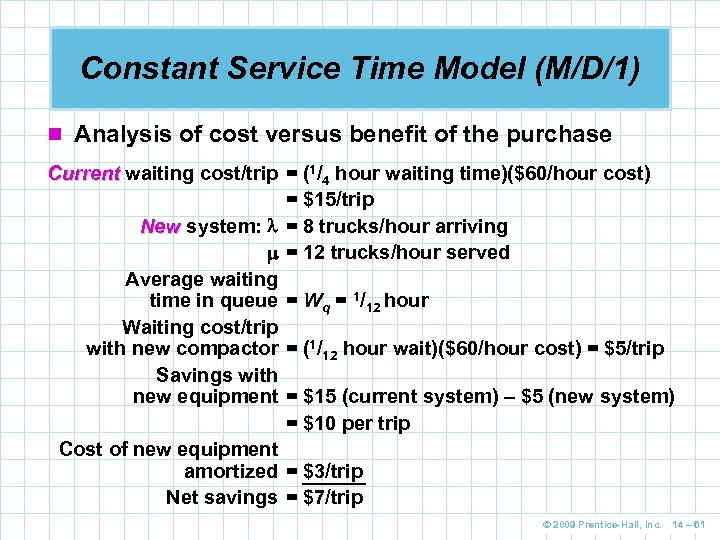Constant Service Time Model (M/D/1) n Analysis of cost versus benefit of the purchase Current waiting cost/trip = (1/4 hour waiting time)(\$60/hour cost) = \$15/trip New system: = 8 trucks/hour arriving = 12 trucks/hour served Average waiting time in queue = Wq = 1/12 hour Waiting cost/trip with new compactor = (1/12 hour wait)(\$60/hour cost) = \$5/trip Savings with new equipment = \$15 (current system) – \$5 (new system) = \$10 per trip Cost of new equipment amortized = \$3/trip Net savings = \$7/trip © 2009 Prentice-Hall, Inc. 14 – 61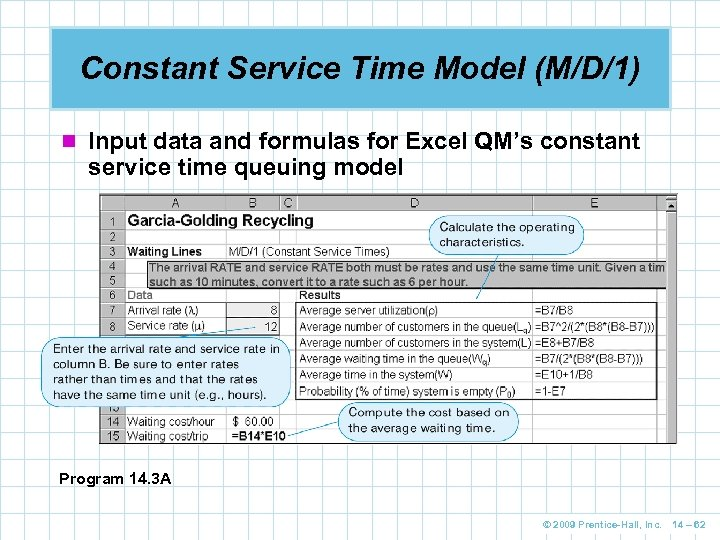Constant Service Time Model (M/D/1) n Input data and formulas for Excel QM’s constant service time queuing model Program 14. 3 A © 2009 Prentice-Hall, Inc. 14 – 62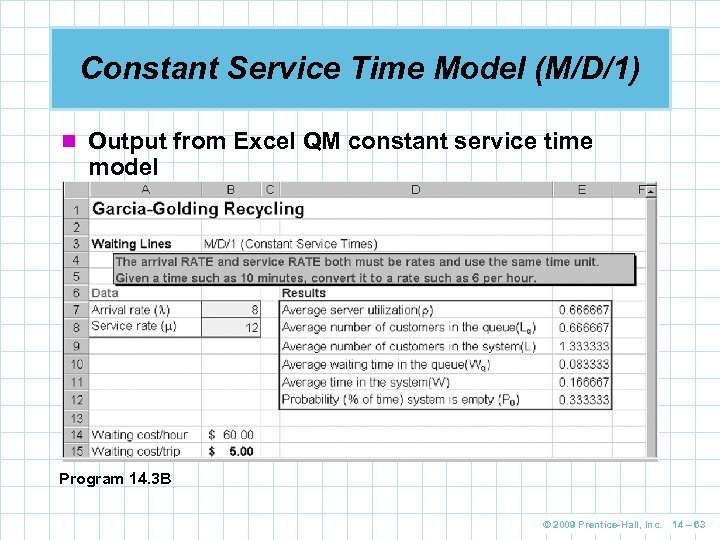Constant Service Time Model (M/D/1) n Output from Excel QM constant service time model Program 14. 3 B © 2009 Prentice-Hall, Inc. 14 – 63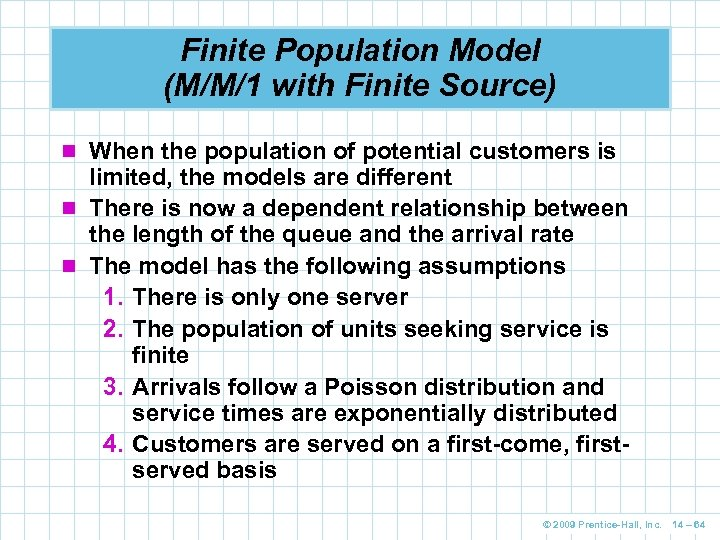Finite Population Model (M/M/1 with Finite Source) n When the population of potential customers is limited, the models are different n There is now a dependent relationship between the length of the queue and the arrival rate n The model has the following assumptions 1. There is only one server 2. The population of units seeking service is finite 3. Arrivals follow a Poisson distribution and service times are exponentially distributed 4. Customers are served on a first-come, firstserved basis © 2009 Prentice-Hall, Inc. 14 – 64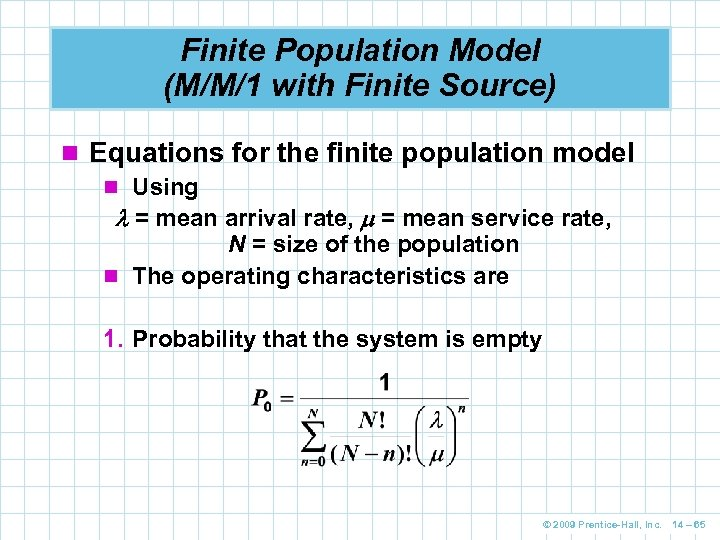Finite Population Model (M/M/1 with Finite Source) n Equations for the finite population model n Using = mean arrival rate, = mean service rate, N = size of the population n The operating characteristics are 1. Probability that the system is empty © 2009 Prentice-Hall, Inc. 14 – 65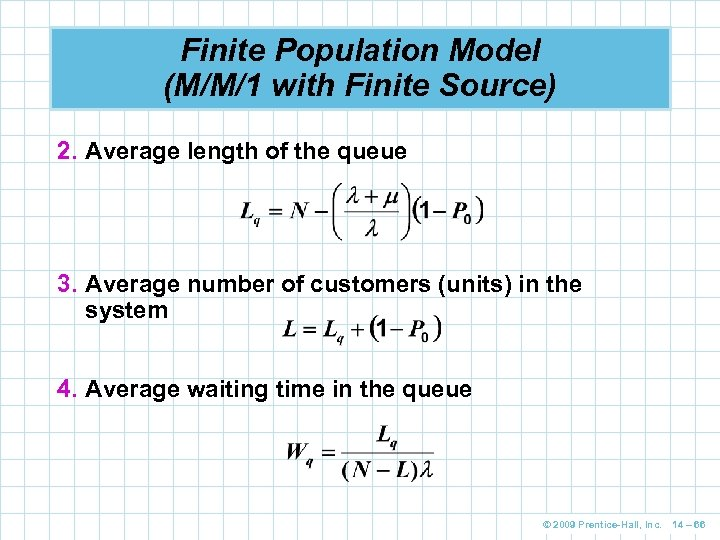Finite Population Model (M/M/1 with Finite Source) 2. Average length of the queue 3. Average number of customers (units) in the system 4. Average waiting time in the queue © 2009 Prentice-Hall, Inc. 14 – 66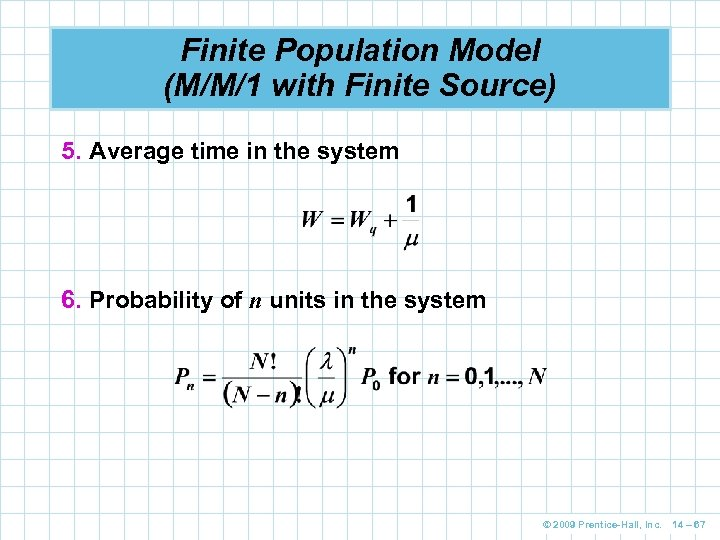Finite Population Model (M/M/1 with Finite Source) 5. Average time in the system 6. Probability of n units in the system © 2009 Prentice-Hall, Inc. 14 – 67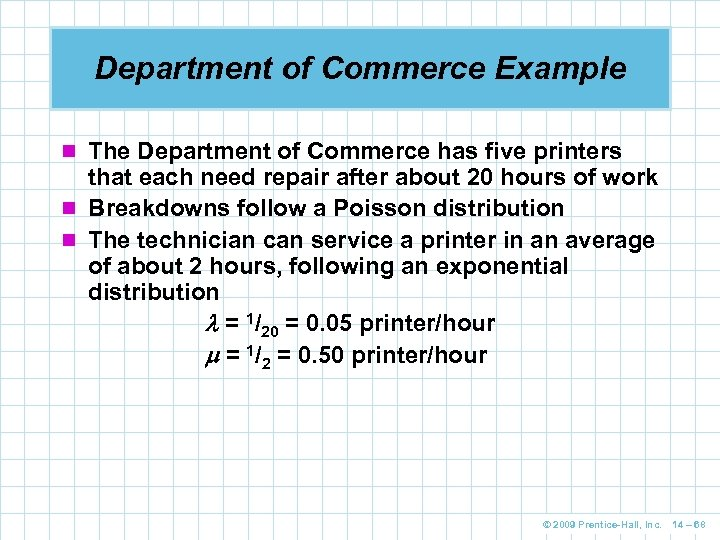Department of Commerce Example n The Department of Commerce has five printers that each need repair after about 20 hours of work n Breakdowns follow a Poisson distribution n The technician can service a printer in an average of about 2 hours, following an exponential distribution = 1/20 = 0. 05 printer/hour = 1/2 = 0. 50 printer/hour © 2009 Prentice-Hall, Inc. 14 – 68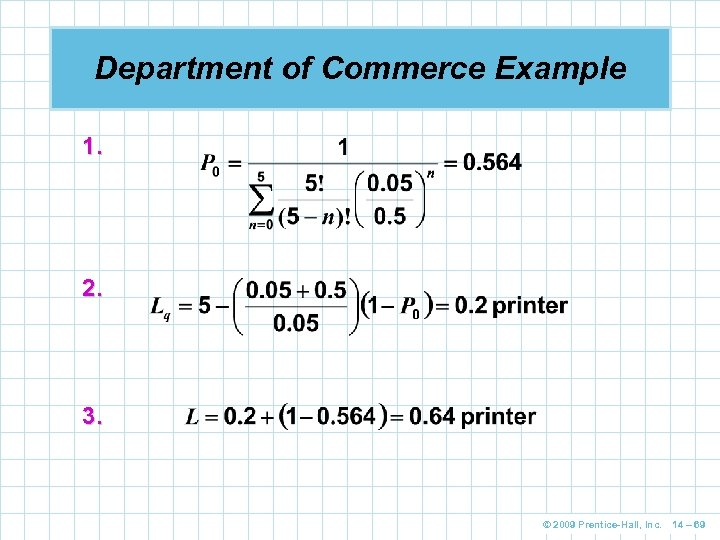Department of Commerce Example 1. 2. 3. © 2009 Prentice-Hall, Inc. 14 – 69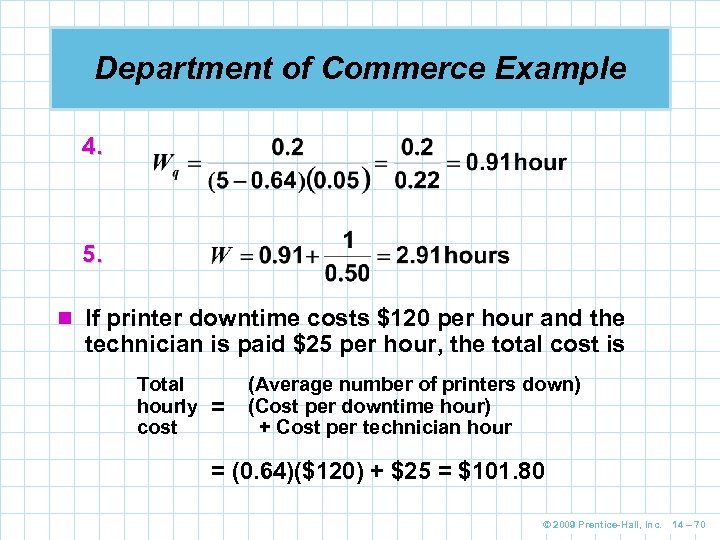Department of Commerce Example 4. 5. n If printer downtime costs \$120 per hour and the technician is paid \$25 per hour, the total cost is Total hourly cost = (Average number of printers down) (Cost per downtime hour) + Cost per technician hour = (0. 64)(\$120) + \$25 = \$101. 80 © 2009 Prentice-Hall, Inc. 14 – 70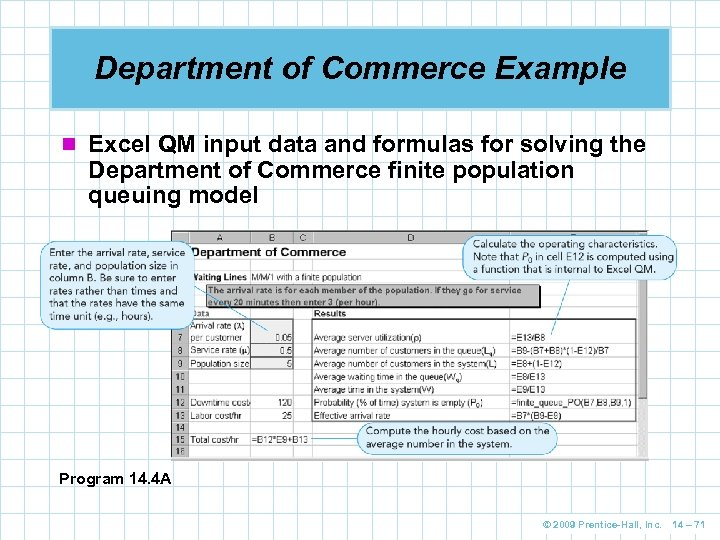Department of Commerce Example n Excel QM input data and formulas for solving the Department of Commerce finite population queuing model Program 14. 4 A © 2009 Prentice-Hall, Inc. 14 – 71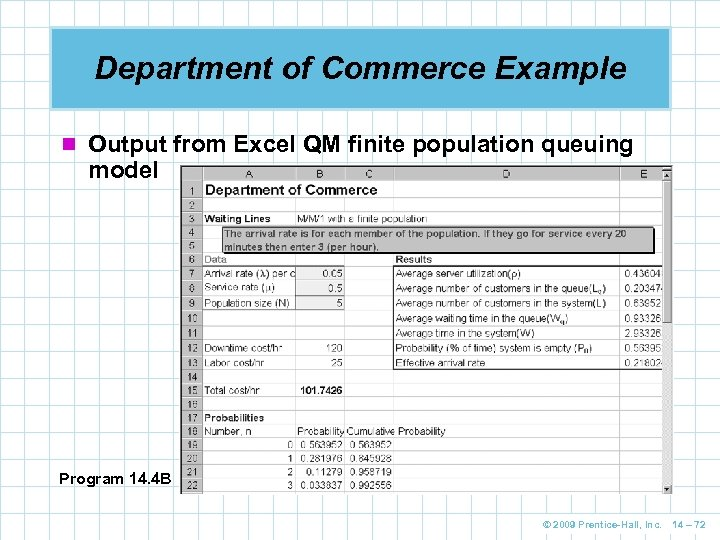Department of Commerce Example n Output from Excel QM finite population queuing model Program 14. 4 B © 2009 Prentice-Hall, Inc. 14 – 72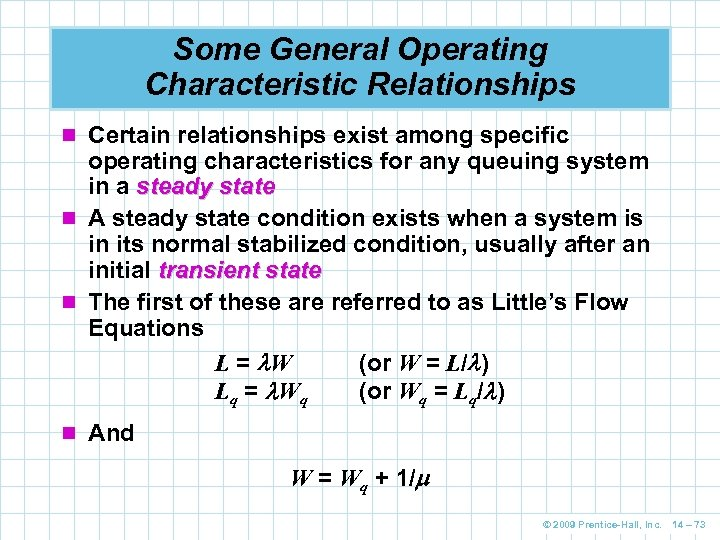Some General Operating Characteristic Relationships n Certain relationships exist among specific operating characteristics for any queuing system in a steady state n A steady state condition exists when a system is in its normal stabilized condition, usually after an initial transient state n The first of these are referred to as Little’s Flow Equations L = W (or W = L/ ) L q = Wq (or Wq = Lq/ ) n And W = Wq + 1/ © 2009 Prentice-Hall, Inc. 14 – 73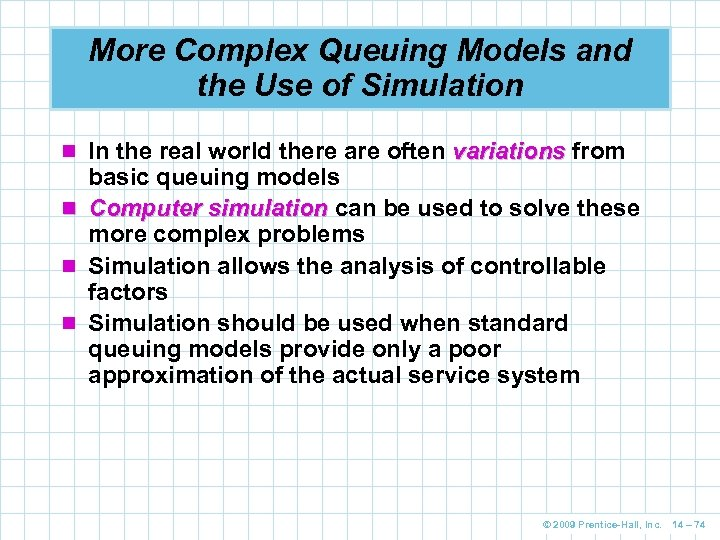More Complex Queuing Models and the Use of Simulation n In the real world there are often variations from basic queuing models n Computer simulation can be used to solve these more complex problems n Simulation allows the analysis of controllable factors n Simulation should be used when standard queuing models provide only a poor approximation of the actual service system © 2009 Prentice-Hall, Inc. 14 – 74